bp神经网络_bp神经网络matlab - CSDN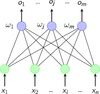bp神经网络 订阅
BP(back propagation)神经网络是1986年由Rumelhart和McClelland为首的科学家提出的概念，是一种按照误差逆向传播算法训练的多层前馈神经网络，是应用最广泛的神经网络。  展开全文BP(back propagation)神经网络是1986年由Rumelhart和McClelland为首的科学家提出的概念，是一种按照误差逆向传播算法训练的多层前馈神经网络，是应用最广泛的神经网络。 

Back Propagation Neural Network

1986年

BP神经网络
BP神经网络发展背景

• 1.1神经网络来源1.2了解感知器认知过程1.3感知器代码实现逻辑或和与1.4感知器网络和S型神经元及激活函数1.5神经网络之结构详解1.6.1神经网络BP算法前置知识1.6.2神经网络BP算法W7过程演练1.6.3神经网络BP算法W1过程...深度学习 深度学习框架 深度学习算法 python深度学习 深度强化学习
• 在之前的文章中，有提到过，所谓的 AI 技术，本质上是一种数据处理处理技术，它的强大来自于两方面：1.互联网的发展带来的海量数据信息 2.计算机深度学习算法的快速发展。 所以说 AI 其实并没有什么神秘，只是在算法...

先向各位小伙伴道歉，文中可能会出现许多错别字，表达不清楚，病句，标点符号使用不当，图片难看且潦草的情况，必须诚恳地向大家表示：凑合看吧，还能咬我咋的...

在之前的文章中，有提到过，所谓的 AI 技术，本质上是一种数据处理处理技术，它的强大来自于两方面：1.互联网的发展带来的海量数据信息  2.计算机深度学习算法的快速发展。 所以说 AI 其实并没有什么神秘，只是在算法上更为复杂。要想理解这一点，我们要从一个问题说起：找数据的规律...

如果你是一名上过大学的人，有几个数学上的方法你应该不太陌生：线性拟合，多项式拟合，最小二乘法...如果这个你都不知道的话，我建议你现在 假装明白 ，然后往下看，应该不难。

先看一下下面这组数据：

x
y
0
1
1
2
2
9
3
28
4
65
5
126
6
217
7
344
8
513
9
730
这里没什么好说的，这个表是一个 x 与 y 的对应关系，我们现在的目标比较明确，找到x与y的对应关系，也就是求y=f(x)的关系式。

第 1 部分  传统数学方法回顾

这部分内容就很简单了，关键记住一个名词 ——  一通(tòng)操作 ...

方法一:线性拟合

我们知道，在大多数情况下，当我们拿来一组数据，进行拟合时，首先想到的肯定是线性拟合，因为其方法简单暴力直接有效，往往很快就能得到一个差不多的结论。虽然不是很精确，但是有句名言说得好，要啥自行车？直接来看结果。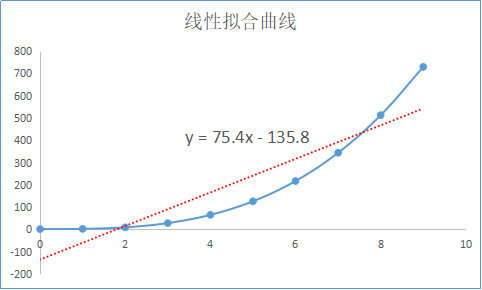关于线性拟合在数学上的方法，这里就不讲了，随便找本教材应该就有，我相信看到上面的图，你应该已经理解了。总之就是经过 一通操作 ，得到线性关系。我这里并没有用数学方法亲自去进行计算和拟合关系式，而是用了一种很高端的工具，叫 Excel ... 以示说明，领会精神即可。

红色虚线为拟合线，蓝色实线为实际点的连线，可以看到，利用线性拟合，得到的结果是 y = 75.4x - 135.8 这样一个数学关系，很显然，它的效果不是他别理想，可以看到，误差还是不小的。

方法二：多项式拟合

这个的思路也比较直接，其实就是假设   ， 比如我们要拟合一个2次的多项式，就可以假设

。同样的，3次方的关系就是 。应该是很好理解吧······

跟刚才一样，又是一通操作，拟合的方法我不就不赘述了，直接用我们的高端工具 Excel 来完成这个工作。效果如下：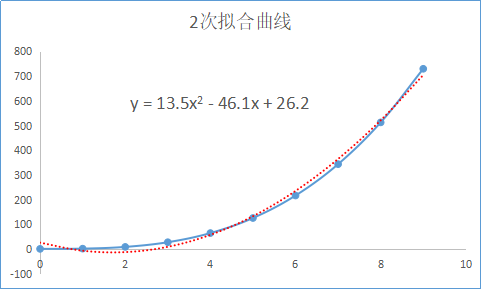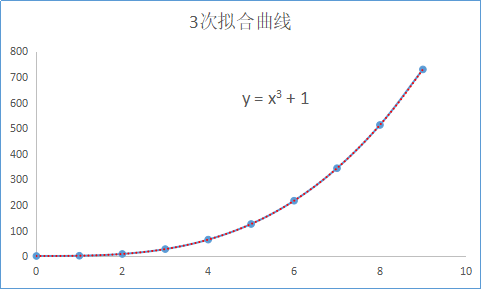可以看到，二次拟合的效果比线性拟合的结果要更接近于真实的结果，而三次曲线就是真实的关系（当然大多数实际情况下并不是严格对应）。

通常利用更高阶的多项式，得到的结果就更加接近于实际的数据。

方法三：其他

最小二乘法，指数拟合，对数拟合，根据数据的不同，用不同的方法来进行拟合得到接近真实情况的数学关系。区别就是利用不同的 一通操作 ... 但是无论是哪一种，解决的数学问题相对来说比较有限，并不能准确拟合出很复杂的数学关系。

如果利用逻辑回归、贝叶斯、决策树、KNN、套袋法等等，也能够解决很多很复杂的数学问题，但这又是另外一个很大的领域，不过建议有机会还是要把这些基础打好，但是这篇博客中，我们不探讨，完全不熟悉也没关系，只要知道这些都是传统的数据处理方法就好。

第 2 部分  现代技术中的难题

下面我们来思考一个 重！要！问！题！：别人爸爸 跟 你爸爸 的不同之处，在数学上的表达是怎么样的 ？

多么深奥的问题，也许你觉得这是一个显而易见的问题，你爸爸就是你爸爸，他爸爸就是他爸爸。这点我十分相信，虽然你不知道如何去回答这个问题，但是你这辈子应该是没喊错过你父亲... 可是问题来了，你怎么让计算机去熟练的分辨出两个人谁是谁？这就必须要依赖数学了...

所以回到问题中来：别人爸爸 跟 你爸爸 的不同之处，在数学上的表达是怎么样的 ？你可能要打我了。但是先别急，先来分析分析。首先，必须明搞明白一件事，这个世界上的事情可以分为两种，可归纳的问题 与 不可归纳的问题。

首先什么是不可归纳的问题，举个例子，你不能用一套完美的数学公式去表达 所有的质数 ， 因为目前的研究表明，还没有什么方法是能够表达质数的，也就是说，质数的出现，本身不具备严格的数学规律，所以无法归纳。

可归纳问题就比较好理解了，一只猫 和 一只狗 出现在你的面前时 ，你能够清晰地将他们进行分辨，这说明在猫和狗之间，确实存在着不同，虽然你很难说清楚它们的不同到底是什么，但是可以知道，这背后是可以通过一套数学表达来完成的，只是很复杂而已。理论上来讲，凡是人类能够掌握的事情，比如再怎么复杂的语言，人类的快速分辨物体的视觉，复杂的逻辑思考，都是可以用数学来表达的可归纳问题。我们人类之所以能够快速地对这些复杂的问题进行快速地反应，得益于我们的大脑内部复杂的神经网络构造。当我们不经意间看到一些物体时，大脑其实是在高速的进行计算，我们天生拥有这种能力，以至于我们根本没有察觉。多么神奇，可以说我们每个人其实都是超级算法工程师...

对比第一部分的那个表格，和如何分辨爸爸的问题，可以得到结论是，这是同一个层次的问题：可归纳数学问题，只是用到的方法不同，复杂度不同而已。都可以用公式来表达：

问题一： x 与 y 的对应关系  y = f(x)

问题二：你爸爸 = f (你爸爸的特征)

这当然是一个复杂的问题，因为首先需要将人的特征转化为数字信息，比如图像（图像本质上就是二位的数组），然后根据不同人的特征，对应的不同人的代号，来拟合一个复杂的，一一对应的函数关系，就是现在技术中的一个难题。解决的方法就是 AI :神经网络。

所以所谓的 AI 技术 ，说到底 是找规律的问题，是拟合复杂函数的问题，是数据处理的问题。

下面将通过实例，来给大家搭建一个最简单的神经网络：Bp神经网络，来理解 AI 。

第 3 部分  最简单的神经网络Bp神经网络

1.Bp 神经网络的简单理解

这里要从名字开始说起了，首先从名称中可以看出，Bp神经网络可以分为两个部分，bp和神经网络。

bp是 Back Propagation 的简写 ，意思是反向传播。而神经网络，听着高大上，其实就是一类相对复杂的计算网络。举个简单的例子来说明一下，什么是网络。

看这样一个问题，假如我手里有一笔钱，N个亿吧(既然是假设那就不怕吹牛逼)，我把它分别投给5个公司，分别占比 M1，M2，M3，M4，M5（M1到M5均为百分比 %）。而每个公司的回报率是不一样的，分别为 A1， A2， A3， A4， A5，（A1到A5也均为百分比 %）那么我的收益应该是多少？这个问题看起来应该是够简单了，你可能提笔就能搞定  收益 = N*M1*A1 + N*M2*A2+N*M3*A3+N*M4*A4+N*M5*A5 。这个完全没错，但是体现不出水平，我们可以把它转化成一个网络模型来进行说明。如下图：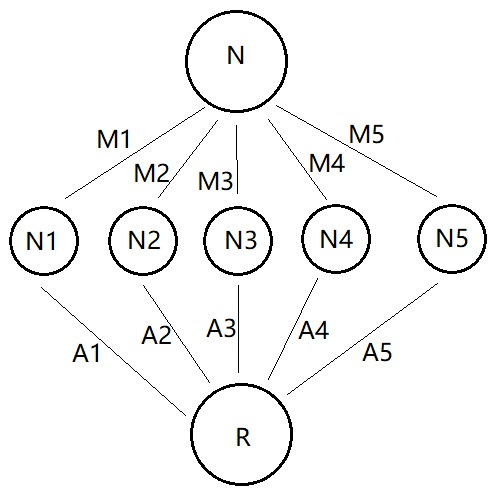图有点丑，领会精神，领会精神。上面的问题是不是莫名其妙的就被整理成了一个三层的网络，N1到N5表示每个公司获得的钱，R表示最终的收益。R = N*M1*A1 + N*M2*A2+N*M3*A3+N*M4*A4+N*M5*A5 。我们可以把 N 作为输入层 ，R作为输出层，N1到N5则整体作为隐藏层，共三层。而M1到M5则可以理解为输入层到隐藏层的权重，A1到A5为隐藏层到输出层的权重。

这里提到了四个重要的概念 输入层（input） ， 隐藏层 （hidden），输出层（output）和权重（weight） 。而所有的网络都可以理解为由这三层和各层之间的权重组成的网络，只是隐藏层的层数和节点数会多很多。

输入层：信息的输入端，上图中 输入层 只有 1 个节点（一个圈圈），实际的网络中可能有很多个

隐藏层：信息的处理端，用于模拟一个计算的过程，上图中，隐藏层只有一层，节点数为 5 个。

输出层：信息的输出端，也就是我们要的结果，上图中，R 就是输出层的唯一一个节点，实际上可能有很多个输出节点。

权重：连接每层信息之间的参数，上图中只是通过乘机的方式来体现。

在上面的网络中，我们的计算过程比较直接，用每一层的数值乘以对应的权重。这一过程中，权重是恒定的，设定好的，因此，是将 输入层N 的 信息 ，单向传播到 输出层R 的过程，并没有反向传播信息，因此它不是神经网络，只是一个普通的网络。

而神经网络是一个信息可以反向传播的网络，而最早的Bp网络就是这一思想的体现。先不急着看Bp网络的结构，看到这儿你可能会好奇，反向传播是什么意思。再来举一个通俗的例子，猜数字：

当我提前设定一个数值 50，让你来猜，我会告诉你猜的数字是高了还是低了。你每次猜的数字相当于一次信息正向传播给我的结果，而我给你的提示就是反向传播的信息，往复多次，你就可以猜到我设定的数值 50 。 这就是典型的反向传播，即根据输出的结果来反向的调整模型，只是在实际应用中的Bp网络更为复杂和数学，但是思想很类似。

2.Bp 神经网络的结构与数学原理（可以不细看）

此节的内容 极！其！重！要！但是要涉及到一些数学，所以我尽量用人话去跟大家细细解释，并且结合实例来给大家进行一下分析。

如果你不想看太多的推导和数学，那么只需要大概理解 Bp 网络的运行思想就好：我们知道，一个函数是由自变量x和决定它的参数θ组成。比如 y=ax + b 中，a，b为函数的固定参数 θ ，x为自变量。那么对于任意一个函数我们可以把它写成 y = f(θ，x)的形式，这里的 θ 代表所有参数的集合[,,,...]，x代表所有自变量的集合[,,,...]。而 Bp 网络的运行流程就是根据已有的 x 与 y 来不停的迭代反推出参数 θ 的过程，这一过程结合了最小二乘法与梯度下降等特殊的计算技巧。这一节看到这儿就基本上可以了，但是如果还想继续深入理解，可以跟着思路，往下接着看。

事实上，这些内容已经被各路神仙们写烂了，因为 Bp网络对于 AI 技术来说，实在太基础，太重要，但是由于在实际学习中，我也遇到过一些困难，现在根据我的学习过程和理解过程，还是要再拿出来写一遍。大神们勿喷···

还是老样子，先来看一个问题，找到下列数据中，y 与 x1，x2，x3的关系，即 y = f（x1，x2，x3）的数学表达式。

表 3.1
x1
x2
x3
y
1
1
2
2
1
2
3
6
2
1
6
12
5
2
5
50
8
3
4
96
7
7
4
196
7
7
7
343
13
8
3
312
6
10
11
660
13
0
17
0
14
7
12
1176
这里一共是 11 组数据（数据量很少），很明显 y 是关于 x1，x2，x3 的三元函数，通常情况下，想要通过一套固定的套路来拟合出一个三元函数的关系式，是一件很复杂的事。而实际问题中的参数往往不止三个，可能成千上百，也就是说 决定 y 的参数会有很多，这样的问题更是复杂的很，用常规的方法去拟合，几乎不可能，那么换一种思路，用 Bp神经网络的方法来试一下。

根据上表给出的条件和问题，我们先来分析一下。首先，我们的输入信息是 3 个参数，x1，x2，x3 。输出结果是 1 个数 y 。那么可以画一个这样的关系网路图（直接手画了，凑合看吧···）：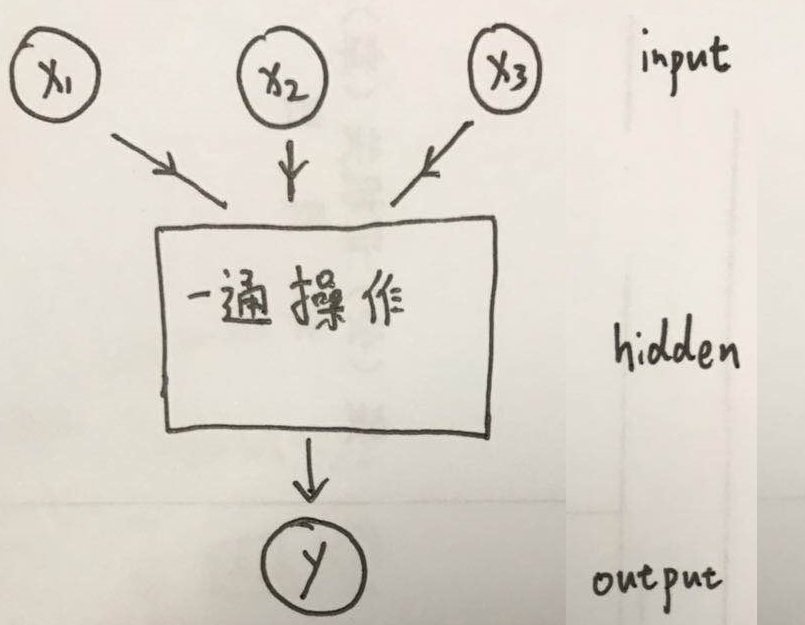在这个网络中，输入层（input ）有三个节点（因为有三个参数），隐藏层（hidden ）先不表示，输出层（output ）有1个节点（因为我们要的结果只有一个 y ）。那么关键的问题来了，如何进行这一通操作，它的结构究竟是怎样的？

2.1 正向传播

正向传播就是让信息从输入层进入网络，依次经过每一层的计算，得到最终输出层结果的过程。

我直接把设计好的结构图给大家画出来，然后再一点一点地解释。结构如下：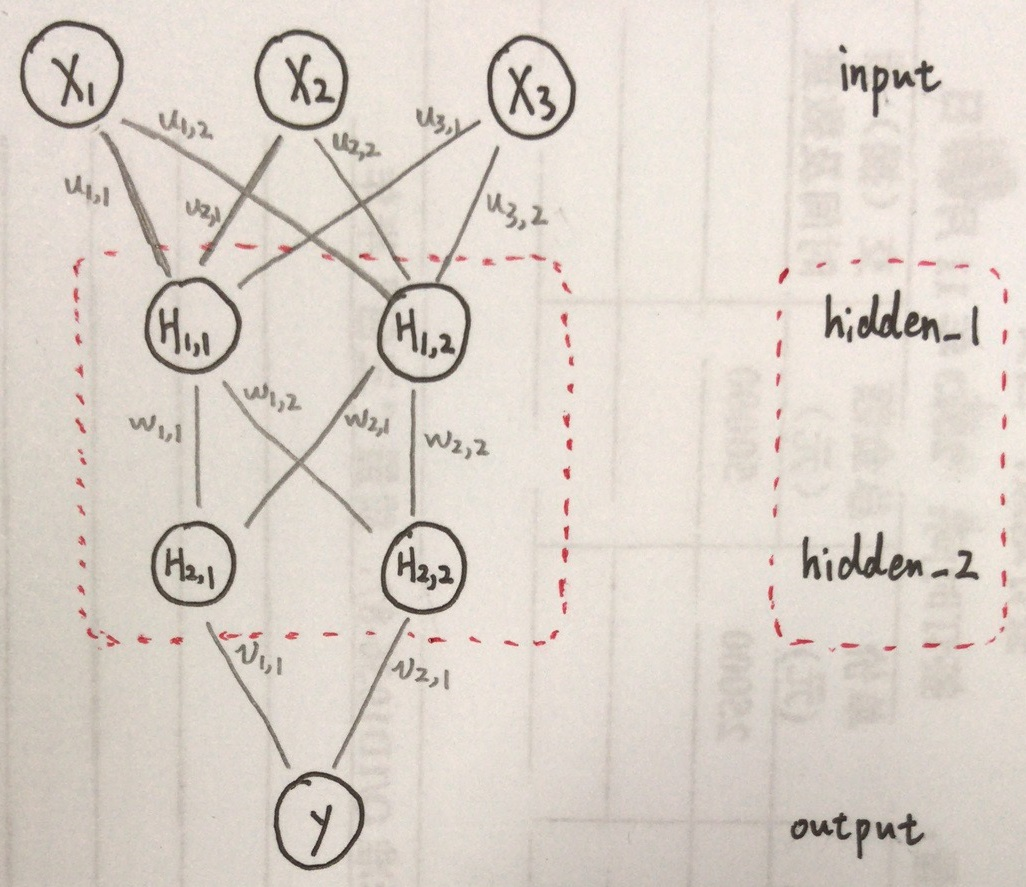看到这儿你可能会有点懵，不过不要紧，一步一步来分析。先来看网络的结构，输入层（input ）没有变，还是三个节点。输出层（input ）也没有变。重点看隐藏层（hidden ），就是图中红色虚线框起的部分，这里我设计了一个隐藏层为两层的网络，hidden_1和hidden_2 ，每层的节点为 2 个，至于为什么是两层，节点数为什么是 2 两个 ，这里你只需要知道，实验证明，解决这个问题，这样的网络就够用了。具体的一会儿讲。

关键看一下连线代表的意义，和计算过程。可以从图上看到，每层的节点都与下一层的每个节点有一一对应的连线，每条连线代表一个权重，这里你可以把它理解为信息传输的一条通路，但是每条路的宽度是不一样的，每条通路的宽度由该通道的参数，也就是该通路的权重来决定。为了说明这个问题，拿一个节点的计算过程来进行说明，看下图：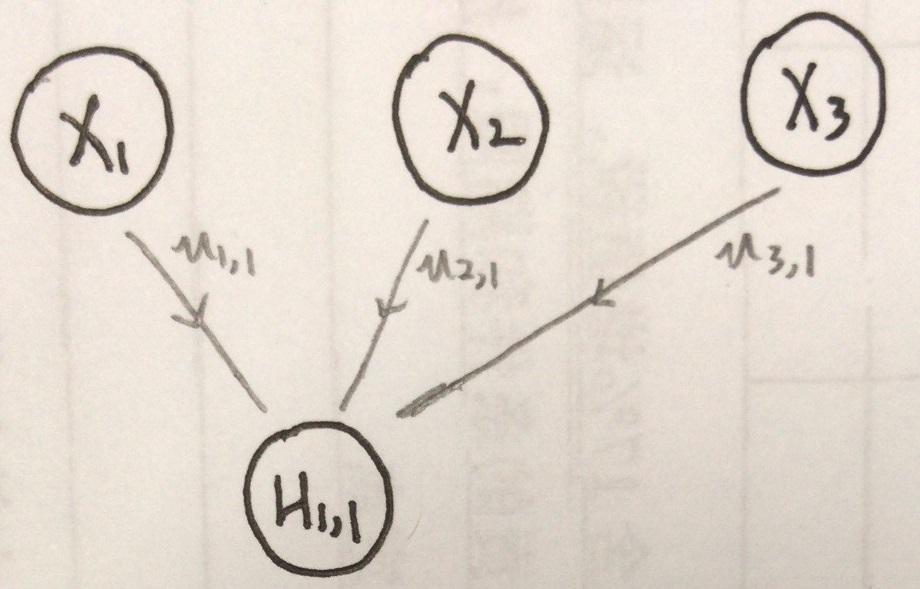这上上图中的一部分，输入层（input ）与 第一层隐藏层（hidden ）的第一个节点 的连接关系。根据上边的图你可能自然的会想到：   。如果你这么想，那就说明你已经开窍了，不过实际过程要复杂一些。我们可以把  这个节点看做是一个有输入，有输出的节点，我们规定输入为  , 输出为  ，则真实的过程如下：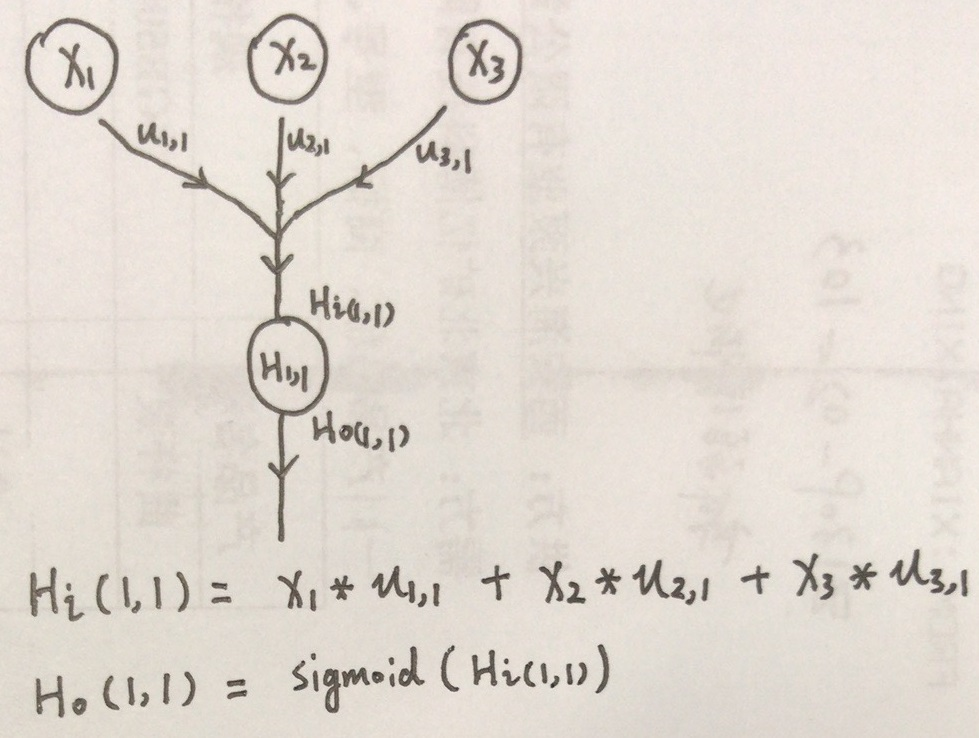计算的方法我直接写到图里了，字儿丑，但是应该能看清楚···解释一下，就是x1，x2，x3与各自权重乘积的和，但是为什么非要搞一个 sigmoid() ，这是什么鬼？ 其实最早人们在设计网络的时候，是没有这个过程的，统统使用线性的连接来搭建网络，但是线性函数没有上界，经常会造成一个节点处的数字变得很大很大，难以计算，也就无法得到一个可以用的网络。因此人们后来对节点上的数据进行了一个操作，利用sigmoid()函数来处理，使数据被限定在一定范围内。此外sigmoid函数的图像是一个非线性的曲线，因此，能够更好的逼近非线性的关系，因为绝大多数情况下，实际的关系是非线性的。sigmoid在这里被称为 激励函数 ，这是神经网络中的一个非常重要的基本概念。下面来具体说一下什么是 sigmoid() 函数。

不作太具体的分析，直接看公式和图像：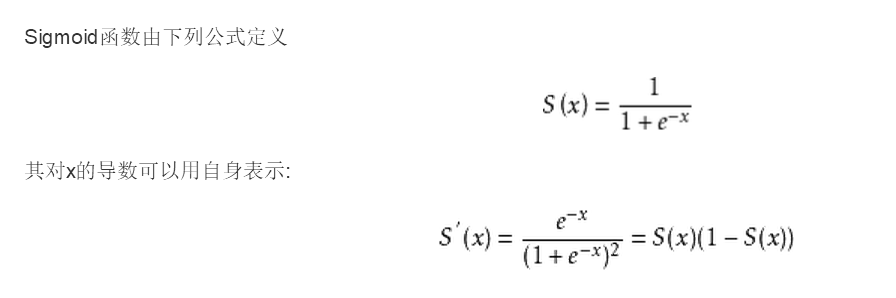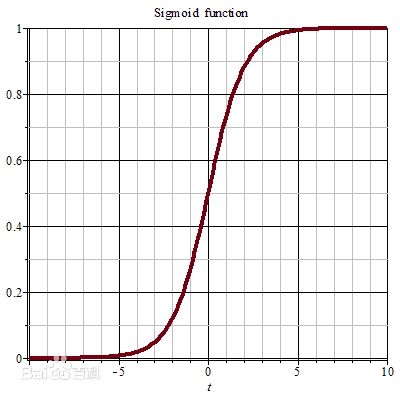图像来自百度百科，可以看到sigmoid函数能够将函数限制在 0到1 的范围之内。

这里还要进行一下说明，sigmoid 是最早使用的激励函数，实际上还有更多种类的激励函数 ，比如 Relu ，tanh 等等，性质和表达式各有不同，以后再说，这里先用 sigmoid 来说明。

如果说看到这儿，你对 激励函数 这个概念还是不太懂的话 ，没关系，可以假装自己明白了，你就知道这个东西很有用，里面必有道道就行了，以后慢慢体会，慢慢理解，就行了。接着往下看。

刚刚解释了一个节点的计算过程，那么其他节点也就可以举一反三，一一计算出来。现在我们来简化一下网络。我们可以把x1，x2，x3作为一个向量 [x1，x2，x3] ,权重矩阵 u 也作为一个 3x2 的矩阵 ，w 作为一个 2x2 的矩阵 ，v作为一个 2x1 的矩阵，三个矩阵如下：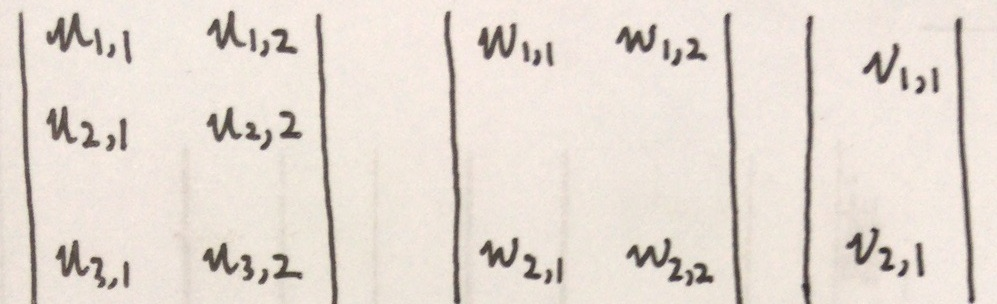可以看到这三个矩阵与网络中的结构图中是一一对应的。下面我们把隐藏层与输出层也写成矩阵的形式：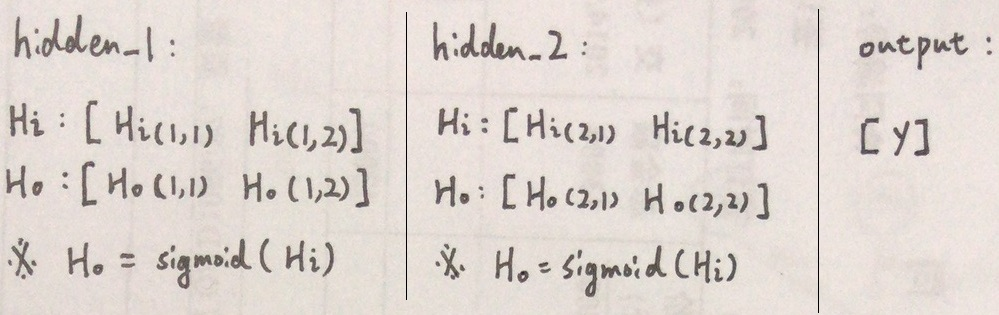可以看到这两层隐藏层（hidden）的输入Hi 与 Ho 均为 1x2 的矩阵，输出层（output ）为 1x1 的矩阵。下面就可以把网络简化为下面的结构：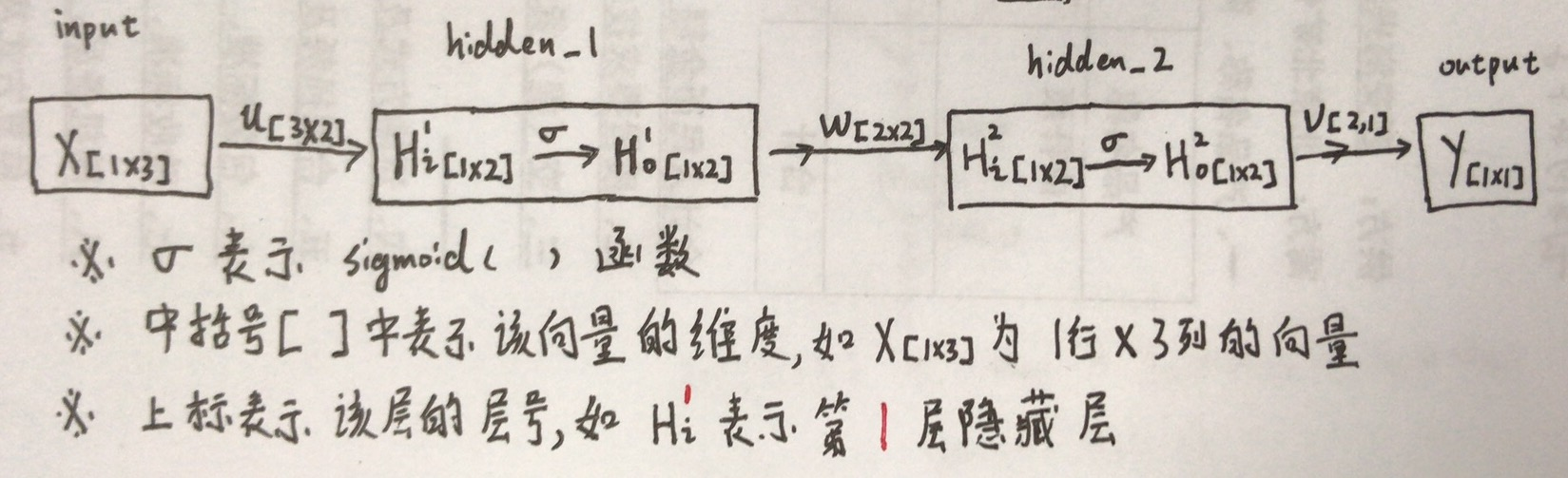根据我们刚才讲过的每个节点的计算方法，以及我们简化后的网络，则可以将整个计算过程等效的化为以下几个矩阵相城的步骤（矩阵相乘是怎么会回事，请复习线性代数...）：

注意：下式中，除sigmoid代表激励函数以外，其余各个符号都代表一个矩阵（或者向量），而非常数，乘积符号“ x ”代表常规的矩阵乘法计算。

※ 由于矩阵 x 维度为 1*3 ，u 维度为 3*2 ，所以自然得到维度为1*2的矩阵（或者向量） 。

※ 维度不变

※ (1*2) x (2*2) →（1*2） 括号内为各矩阵维度

※ 维度不变

※ (1*2) x (2*1) →（1*1）  括号内为各矩阵维度

注意：细心的小伙伴应该发现公式中出现了几个之前没有提到的符号 ，， 。它们也各自代表一个矩阵，它们的概念为阈值，通常用符号b来表示。阈值的意义是，每个节点本身就具有的一个数值，设置阈值能够使网络更快更真实的去逼近一个真实的关系。

以上这个过程，就是该网络的信息进行了一次 正向传播 。

2.2 反向传播

那么有正向传播，就必须得有反向传播，下面来讲一下 反向传播 的过程。首先明确一点，反向传播的信息是什么，不卖关子，直接给答案，反向传播的信息是误差，也就是 输出层（output ）的结果 与 输入信息 x 对应的真实结果 之间的差距（表达能力比较差，画个图说明...）。

拿出上文的数据表中的第一组数据  x1 = 1，x2=1，x3=2，y=2 为例。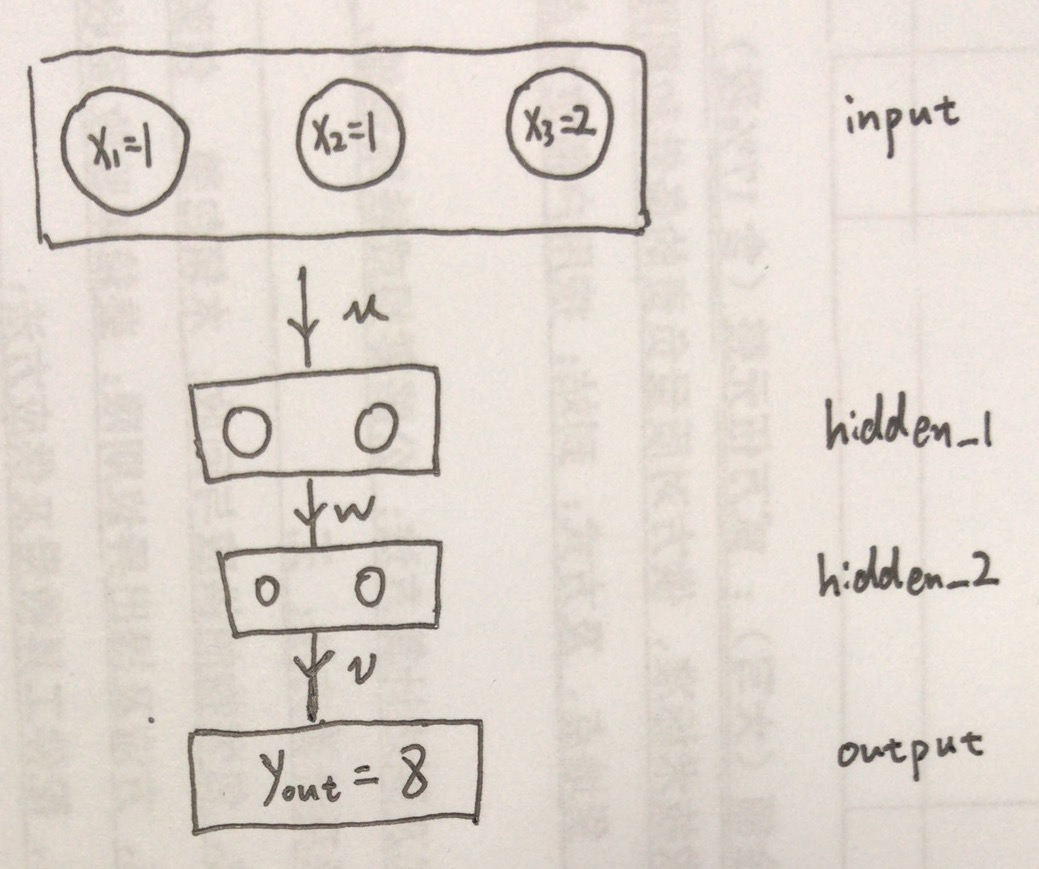假设我们将信息x1，x2，x3 输入给网络，得到的结果为  = 8 ，而我们知道真实的 y 值为 2，因此此时的误差为 |-y|  ，也就是 6 。 真实结果与计算结果的误差被称作 损失 loss ， loss = | - y|  记作 损失函数 。这里有提到了一个很重要的概念，损失函数，其实在刚才的例子中，损失函数 loss = | - y|  只是衡量误差大小的一种方式，称作L1损失（先知道就行了），在实际搭建的网络中，更多的用到的损失函数为 均方差损失，和交叉熵损失。原则是分类问题用交叉熵，回归问题用均方差，综合问题用综合损失，特殊问题用特殊损失···以后慢慢说吧，因为损失函数是一个超级庞大的问题。

总之我们先知道，损失函数 loss 是一个关于 网络输出结果  与真实结果 y 的，具有极小值的函数 。那么我们就可以知道，如果一个网络的计算结果  与 真是结果 y 之间的损失总是很小，那么就可以说明这个网络非常的逼近真实的关系。所以我们现在的目的，就是不断地通过调整权重u，w，v（也就是网络的参数）来使网络计算的结果  尽可能的接近真实结果 y ，也就等价于是损失函数尽量变小。那么如何调整u，w，v 的大小，才能使损失函数不断地变小呢？这理又要说到一个新的概念：梯度下降法 。

梯度下降法 是一个很重要很重要的计算方法，要说明这个方法的原理，就又涉及到另外一个问题：逻辑回归。为了简化学习的过程，不展开讲，大家可以自己去搜一下逻辑回归，学习一下。特别提醒一下，逻辑回归是算法工程师必须掌握的内容，因为它对于 AI 来说是一个很重要的基础。下面只用一个图(图片来自百度)进行一个简单地说明。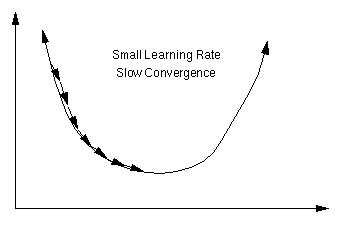假设上图中的曲线就是损失函数的图像，它存在一个最小值。梯度是一个利用求导得到的数值，可以理解为参数的变化量。从几何意义上来看，梯度代表一个损失函数增加最快的方向，反之，沿着相反的方向就可以不断地使损失逼近最小值，也就是使网络逼近真实的关系。

那么反向传播的过程就可以理解为，根据 损失loss ，来反向计算出每个参数（如  , 等）的梯度 d() ，d() ....等等，再将原来的参数分别加上自己对应的梯度，就完成了一次反向传播。

来看看 损失loss 如何完成一次反向传播，这里再定义一些变量  ，  和  。注意：它们都代表矩阵（向量），而非一个数值。它们分别代表第一层，第二层隐藏层，以及输出层每个神经元节点反向输出的值。 分别代表权值矩阵与阈值矩阵对应的梯度矩阵,用符号  代表损失，来表示sigmoid函数的导数。这里只简单的说一下计算公式，推导过程后边讲。

计算梯度，注意：下式中未标红的都代表一个矩阵（或者向量），标红符号的代表一个常数。

更新权值与阈值

※ 公式中的 乘号 “ x ”表示常规的矩阵乘积运算，运算后会发生维度的变化。 符号 “ · ” 表示按位乘积，运算后维度不变。

以上就是一次完整的反向传播过程，需要说明的是，上式当中用到了一个符号  ，这又是一个重要的概念，学习率，一个小于1的实数，它的大小会影响网络学习的速率以及准确度。可以把它理解为梯度下降时的步长。

反向传播过程实际上还是有点复杂的，下面我来简单说一下为什么梯度是这样求的。

我们知道，整个网络可以简化成一个函数 ，也就是说这个函数的表达式，主要由各个参数  来决定，而现在为了确定网络的参数，则可以把  作为函数的自变量，而x作为参数，对  求偏导     ，这个偏导的结果就是该参数  对应的梯度，这个思想实际上来自于最小二乘法，反正求完就是上边式子中的结果，这里不再进行推导。

2.3 网络的训练

通过一次正向传播，和一次反向传播，我们就可以将网络的参数更新一次，所谓训练网络，就是让正向传播和反向传播不断的往复进行，不断地更新网络的参数，最终使网络能够逼近真实的关系。

理论上，只要网络的层数足够深，节点数足够多，可以逼近任何一个函数关系。但是这比较考验你的电脑性能，事实上，利用 Bp 网络，能够处理的数据其实还是有限的，比如 Bp 网络在图像数据的识别和分类问题中的表现是很有限的。但是这并不影响 Bp 网络是一种高明的策略，它的出现也为后来的 AI 技术做了重要的铺垫。

3.Bp 神经网络的代码实现

回到 表 3.1 中的数据，将用 python 来实现一个 Bp 网络 ，对数据的关系建立一个网络模型。

这里有几点需要说明，首先在数据进入网络之前，要先进行归一化处理，即将数据除以一个数，使它们的值都小于 1 ，这样做的目的是避免梯度爆炸。其次为了更好、更快的收敛得到准确的模型，这里采用了对数据进行特征化的处理。最后，这段代码中用到的激励函数是Relu，并非我们之前所讲的 sigmoid ，因为Relu的计算速度更快，更容易收敛。

这里有几个参数和数组需要说明，其中 p_s 中的数组代表 表 3.1 中 11组数据的 [x1，x2，x3] ，t_s代表对应的 y 。p_t 与t_t用来存放测试网络训练效果的 测试数据集 。我们用p_s与t_s来训练 Bp 网络 ，用 p_t 与 t_t 来检验训练的效果。表 3.1 的数据中，y 与 x1，x2，x3 的对应关系实际上是 y = x1 * x2 * x3 。

代码如下：

import time
from numpy import *

######## 数据集 ########

p_s = [[1,1,2],[1,2,3],[2,1,6],[5,2,5],[8,3,4],[7,7,4],[7,7,7],[13,8,3],[6,10,11],[13,0,17],[14,7,12]]              # 用来训练的数据集 x
t_s = [,,,,,,,,,,]   # 用来训练的数据集 y

p_t = [[6,9,1017],[2,3,4],[5,9,10]]      # 用来测试的数据集 x_test
t_t = [,,]               # 用来测试的数据集 对应的实际结果 y_test

######## 超参数设定 ########

n_epoch = 20000             # 训练次数

HNum = 2;                   # 各层隐藏层节点数

HCNum = 2;                  # 隐藏层层数

AFKind = 3;                 # 激励函数种类
emax = 0.01;                # 最大允许均方差根
LearnRate = 0.01;           # 学习率

######## 中间变量设定 ########
TNum = 7;                   # 特征层节点数 (特征数)

SNum = len(p_s);            # 样本数

INum = len(p_s);         # 输入层节点数（每组数据的维度）
ONum = len(t_s);         # 输出层节点数（结果的维度）
StudyTime = 0;              # 学习次数
KtoOne = 0.0;               # 归一化系数
e = 0.0;                    # 均方差跟

######################################################### 主要矩阵设定 ######################################################

I = zeros(INum);

Ti = zeros(TNum);
To = zeros(TNum);

Hi = zeros((HCNum,HNum));
Ho = zeros((HCNum,HNum));

Oi = zeros(ONum);
Oo = zeros(ONum);

Teacher = zeros(ONum);

u = 0.2*ones((TNum,HNum))                  # 初始化 权值矩阵u
w = 0.2*ones(((HCNum-1,HNum,HNum)))        # 初始化 权值矩阵w
v = 0.2*ones((HNum,ONum))                  # 初始化 权值矩阵v

dw = zeros((HCNum-1,HNum,HNum))

Hb = zeros((HCNum,HNum));
Ob = zeros(ONum);

He = zeros((HCNum,HNum));
Oe = zeros(ONum);

p_s = array(p_s)
t_s = array(t_s)
p_t = array(p_t)

################################# 时间参数 #########################################

time_start = 0.0
time_gyuyihua = 0.0
time_nnff = 0.0
time_nnbp = 0.0
time_begin = 0.0

time_start2 = 0.0

time_nnff1 = 0.0
time_nnff2 = 0.0
time_nnbp_v = 0.0
time_nnbp_w = 0.0
time_nnbp_u = 0.0
time_nnbp_b = 0.0

######################################################### 方法 #######################################################

def Calcu_KtoOne(p,t):                         # 确定归一化系数
p_max = p.max();
t_max = t.max();
return max(p_max,t_max);

def trait(p):                                  # 特征化
t = zeros((p.shape,TNum));
for i in range(0,p.shape,1):
t[i,0] = p[i,0]*p[i,1]*p[i,2]
t[i,1] = p[i,0]*p[i,1]
t[i,2] = p[i,0]*p[i,2]
t[i,3] = p[i,1]*p[i,2]
t[i,4] = p[i,0]
t[i,5] = p[i,1]
t[i,6] = p[i,2]

return t

def AF(p,kind):   # 激励函数
t = []
if kind == 1:   # sigmoid
pass
elif kind == 2:   # tanh
pass
elif kind == 3:    # ReLU

return where(p<0,0,p)
else:
pass

def dAF(p,kind):   # 激励函数导数
t = []
if kind == 1:   # sigmoid
pass
elif kind == 2:   # tanh
pass
elif kind == 3:    # ReLU

return where(p<0,0,1)
else:
pass

def nnff(p,t):
pass

def nnbp(p,t):
pass

def train(p,t):                                # 训练

global e
global v
global w
global dw
global u
global I
global Ti
global To
global Hi
global Ho
global Oi
global Oo
global Teacher
global Hb
global Ob
global He
global Oe
global StudyTime
global KtoOne

global time_start
global time_gyuyihua
global time_nnff
global time_nnbp
global time_start2
global time_nnff1
global time_nnff2
global time_nnbp_v
global time_nnbp_w
global time_nnbp_u
global time_nnbp_b

time_start = time.clock()

e = 0.0
p = trait(p)

KtoOne = Calcu_KtoOne(p,t)

time_gyuyihua += (time.clock()-time_start)

time_start = time.clock()

for isamp in range(0,SNum,1):
To = p[isamp]/KtoOne
Teacher = t[isamp]/KtoOne

################ 前向 nnff #############################

time_start2 = time.clock()
######## 计算各层隐藏层输入输出 Hi Ho ########

for k in range(0,HCNum,1):
if k == 0:
Hi[k] = dot(To,u)
else:
Hi[k] = dot(Ho[k-1],w[k-1])

time_nnff1 += (time.clock()-time_start2)
time_start2 = time.clock()

########   计算输出层输入输出 Oi Oo    ########
Oi = dot(Ho[HCNum-1],v)

time_nnff2 += (time.clock()-time_start2)
time_start2 = time.clock()
time_nnff += (time.clock()-time_start)
time_start = time.clock()

################ 反向 nnbp #############################

######## 反向更新 v ############

Oe = subtract(Teacher,Oo)

e += sum(multiply(Oe,Oe))

#### v 梯度 ####

dv = dot(array([Oe]),array([Ho[HCNum-1]])).transpose()			  # v 的梯度

v = add(v,dv*LearnRate)    # 更新 v

time_nnbp_v += (time.clock()-time_start2)

time_start2 = time.clock()

######## 反向更新 w #############
He = zeros((HCNum,HNum))

for c in range(HCNum-2,-1,-1):
if c == HCNum-2:
He[c+1] = dot(v,Oe)

#dw[c] = dot(array([He[c+1]]),array([Ho[c]]).transpose())
dw[c] = dot(array([Ho[c]]).transpose(),array([He[c+1]]))
#dw[c] = dw[c].transpose()  #@@@@@@ 若结果不理想，可尝试用此条语句

else:
He[c+1] = dot(w[c+1],He[c+2])

dw[c] = dot(array([Ho[c]]).transpose(),array([He[c+1]]))

time_nnbp_w += (time.clock()-time_start2)

time_start2 = time.clock()

######## 反向更新 u #############

He = dot(w,He)

du = dot(array([To]).transpose(),array([He]))

time_nnbp_u += (time.clock()-time_start2)

time_start2 = time.clock()

######### 更新阈值 b ############

Ob = Ob + Oe*LearnRate

Hb = Hb + He*LearnRate

time_nnbp += (time.clock()-time_start)

time_start = time.clock()

time_nnbp_b += (time.clock()-time_start2)

time_start2 = time.clock()

e = sqrt(e)

def predict(p):

p = trait(p)
p = p/KtoOne
p_result = zeros((p.shape,1))

for isamp in range(0,p.shape,1):
for k in range(0,HCNum,1):
if k == 0:
Hi[k] = dot(p[isamp],u)
else:
Hi[k] = dot(Ho[k-1],w[k-1])

########   计算输出层输入输出 Oi Oo    ########
Oi = dot(Ho[HCNum-1],v)
Oo = Oo*KtoOne
p_result[isamp] = Oo
return p_result

time_begin = time.clock()

for i in range(1,n_epoch,1):
if i%1000 == 0:
print('已训练 %d 千次 ,误差均方差 %f'%((i/1000),e))
train(p_s,t_s)
print('训练完成，共训练 %d 次，误差均方差 %f'%(i,e))

print('共耗时: ',time.clock()-time_begin)

print()

result = predict(p_t)

print('模型预测结果 : ')
for i in result:
print('%.2f'%i)

print('\n实际结果 : ')
for i in t_t:
print(i)



运行代码后，得到的结果如下图：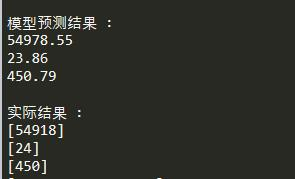可以看到，经过训练后，该 Bp 网络确实从原始数据中学到了特征 ， 并且较为准确地对测试数据进行了推测。

此外还要说明，此段代码历史较为悠久，因此很多地方写的很不规范（很多地方保持了C的习惯···实际上是多余的），符号使用的也比较混乱（但是实在懒得整理），仅拿来供大家参考和理解，望小伙伴们见谅。

4.Bp 神经网络的经验总结

以上内容对 Bp 网络的基本用法和数学关系 进行了讲解。下面有几个重要的知识点，需要特别指出：

a.对于一个神经网络来说，更宽更深的网络，能够学到更加复杂的特征，其能够解决的问题也就越复杂，但是其计算过程也越繁琐，参数越多，越容易出现过拟合的情况（过拟合即网络过度学习了数据的特征，将噪声也同时考虑到了网络中，造成网络只在训练集上表现良好，而无法泛化到其他数据上，说白了就是这个网络已经学傻了...），因此要根据数据的实际情况来设计网络的层数，节点数，激励函数类型 以及 学习率。

b.对于一个神经网络来说，用来训练神经网络的数据集的质量，很大程度上决定了网络的预测效果。数据越丰富，神经网络越能够贴近实际关系，泛化能力越强。

c.Bp神经网络是区别于传统数据处理的一种方法，其特点在于寻找数据之间的相关性，并非严格地数学关系，因此是一种有效但是并非严格地网络。对于实际问题的处理非常有用，但不能作为严谨数学计算的方法。

Bp网络的出现，为后来的 AI 技术提供了理论基础，无论是 AlphaGo ，计算机视觉，还是自然语言处理等复杂问题，都可以理解为这一结构的升级和变种（不过升级幅度有点大，变化样式有点多···）。因此这一对于这一网络的理解，大家应该亲自写写代码，多看一看大神们写的推导过程，深入理解。
展开全文BP神经网络 人工智能
• ## 详解BP神经网络

万次阅读 多人点赞 2020-09-18 00:57:12
BP神经网络学习算法可以说是目前最成功的神经网络学习算法。显示任务中使用神经网络时，大多数是使用BP算法进行训练.   在我看来BP神经网络就是一个”万能的模型+误差修正函数“，每次根据训练得到的结果与...
#BackPropagation Neuron NetWok
BP神经网络学习算法可以说是目前最成功的神经网络学习算法。显示任务中使用神经网络时，大多数是使用BP算法进行训练.
在我看来BP神经网络就是一个”万能的模型+误差修正函数“，每次根据训练得到的结果与预想结果进行误差分析，进而修改权值和阈值，一步一步得到能输出和预想结果一致的模型。举一个例子：比如某厂商生产一种产品，投放到市场之后得到了消费者的反馈，根据消费者的反馈，厂商对产品进一步升级，优化，从而生产出让消费者更满意的产品。这就是BP神经网络的核心。
下面就让我们来看看BP算法到底是什么东西。BP网络由输入层、隐藏层、输出层组成。给定训练集***D***={(x1,y1),(x2,y2…(xn,yn)},其中xnϵRd，ynϵRl,表示输入示例由d个属性组成，输出l维实值变量。现在，我们看看如何求得输出值，以及怎么由输出值调整权值和阈值。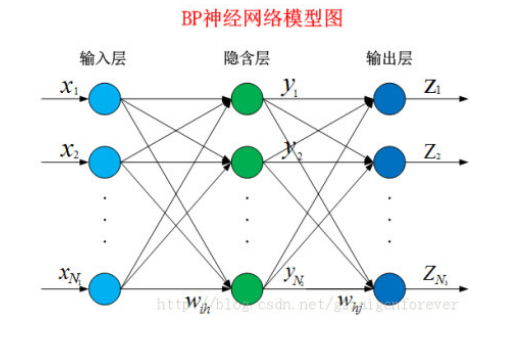神经元是以生物研究及大脑的响应机制而建立的拓扑结构网络，模拟神经冲突的过程，多个树突的末端接受外部信号，并传输给神经元处理融合，最后通过轴突将神经传给其它神经元或者效应器。神经元的拓扑结构如图：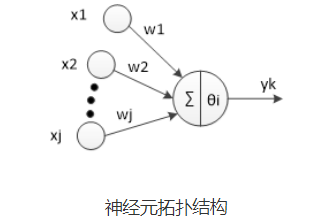对于第i个神经元，X1、X2、…、Xj为神经元的输入，输入常为对系统模型关键影响的自变量，W1、W2、…、Wj为连接权值调节各个输入量的占重比。将信号结合输入到神经元有多种方式，选取最便捷的线性加权求和可得neti神经元净输入:
$Net_{in}=\sum_{i=1}^{n}{w_i*x_i}$
$\theta$i表示该神经元的阈值，根据生物学中的知识，只有当神经元接收到的信息达到阈值是才会被激活。因此，我们将$Net_{in}$和$\theta_j$进行比较，然后通过激活函数处理以产生神经元的输出。
激活函数：激活函数这里我们不多重述。如果输出值有一定的范围约束，比如用来分类，一般我们用的最多的是Sigmod函数，它可以把输入从负无穷大到正无穷大的信号变换成0到1之间输出。如果没有约束的话，我们可以使用线性激活函数(即权值相乘之和)。这样我们得到的输出为：
$y_j=f(Net_{in}-\theta_j)$
我们可以将公式化简一下，设第一个输入永远值为$\theta$,权值为-1，则我们可以得到公式：
$y_j=f(\sum_{i=0}^nw_i*x_i)$
其中w0=-1,x0=$\theta$j,其中f为选择的激活函数。
已经知道在BP神经网络模型中，我们有三层结构，输入层、隐藏层、输出层,因此输入层到隐藏层的权值，设为$v_{ih}$,隐藏层第h个神经元的阈值我们设为$\gamma_h$。隐藏层到输出层的权值，设为$w_{hj}$,输出层第j个神经元的阈值我们用$\theta_j$表示。在下面这张图里，有d输入神经元,q个隐藏神经元，隐藏有q个隐藏神经元阈值，$l$个输出神经元，因此有$l$个输出神经元阈值。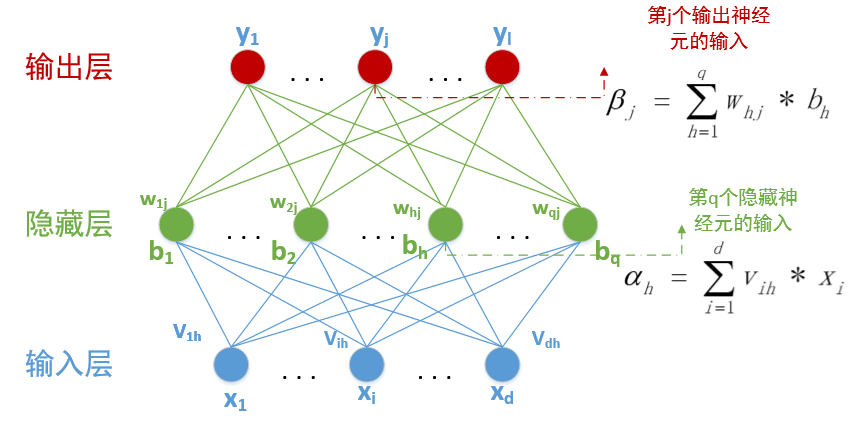其中$\beta_j$中的$b_h=f(\alpha_h-\gamma_h)$。隐藏层和输出层的激活函数，在这里我们暂时全部用$Sigmod$函数。
在某个训练示例$(x_k,y_k)$中，假设神经网络的训练输出为$y_{k^,}=({y_1^{k^,},y_2^{k^,},\cdots,y_l^{k^,}})$,输出为$l$维向量，其中
$y_i^{k^,}=f(\beta_i-\theta_i)$
那么这次预测结果的误差我们可以用最小二乘法表示：
$E_k=\frac{1}{2}\sum_{j=1}^l(y_j^{k^,}-y_j^k)^2$
而我们现在要做的就是根据这个误差去调整$（d+l+1）q+l$个参数的值，一步一步缩小$E_k$。那么从现在开始，我们就要进入数学的世界了。这里我们使用最常用的算法：梯度下降法来更新参数。函数永远是沿着梯度的方向变化最快，那么我们对每一个需要调整的参数求偏导数，如果偏导数>0,则要按照偏导数相反的方向变化；如果偏导数<0，则按照此方向变化即可。于是我们使用-1*偏导数则可以得到参数需要变化的值。同时我们设定一个学习速率$\eta$，这个学习速率不能太快，也不能太慢。太快可能会导致越过最优解；太慢可能会降低算法的效率。(具体设多少就属于玄学调参的领域了)。因此我们可以得到一个参数调整公式：
$Param+=-\eta\frac{\partial E_k}{\partial Param}$
首先我们看看隐藏层到输出层的权值调整值：
$\Delta w_{hj}=-\eta\frac{\partial E_k}{\partial w_{hj}}$
好，我们从上到下缕一缕这个偏导该怎么求，我们把每一个公式都罗列出来：
#####  1.输入层到隐藏层：
$\alpha_h=\sum_{i=1}^dv_{ih}*x_i \cdots \cdots \cdots \cdots \cdots \cdots \cdots(1)$
$\begin{vmatrix} x_1 &x_2 &x_3&\cdots& x_d \end{vmatrix} \cdot \begin{vmatrix} v_{11} &v_{12} &v_{13}& \cdots& v_{1q} \\ v_{21} &v_{22} &v_{23}& \cdots& w_{2q} \\ \cdot & \cdot &\cdot & \cdot &\cdot \\ \cdot & \cdot &\cdot & \cdot &\cdot \\ \cdot & \cdot &\cdot & \cdot &\cdot \\ v_{d1} &w_{d2}&w_{d3} &\cdots & w_{dq} \end{vmatrix}$
#####  2.经过隐藏层的激活函数：
$b_h=f(\alpha_h-\gamma_h) \cdots \cdots \cdots \cdots \cdots \cdots \cdots(2)$
#####  3.隐藏层到输出层：
$\beta_j=\sum_{h=1}^qw_{hj}*b_h \cdots\cdots \cdots \cdots \cdots \cdots \cdots(3)$
#####  用矩阵表示
$\begin{vmatrix} b_1 &b_2 &b_3&\cdots& b_q \end{vmatrix} \cdot \begin{vmatrix} w_{11} &w_{12} &w_{13}& \cdots& w_{1l} \\ w_{21} &w_{22} &w_{23}& \cdots& w_{2l} \\ \cdot & \cdot &\cdot & \cdot &\cdot \\ \cdot & \cdot &\cdot & \cdot &\cdot \\ \cdot & \cdot &\cdot & \cdot &\cdot \\ w_{q1} &w_{q2}&w_{q3} &\cdots & w_{ql} \end{vmatrix}$
#####  4.经过输出层的激活函数：
$y_j^{k^,}=f(\beta_j-\theta_j)\cdots \cdots \cdots \cdots \cdots \cdots \cdots(4))$
#####  5.误差：
$E_k=\frac{1}{2}\sum_{j=1}^l(y_j^{k^,}-y_j^k)^2 \cdots \cdots \cdots \cdots \cdots \cdots \cdots(5)$
综上我们可以得知$w_{hj}$先影响$\beta_j$,再影响$y_j^{k^,}$,最后影响$E_k$,(一个$w$权值只会影响一个$\beta$)所以我们可得：
$\Delta w_{hj}=-\eta\frac{\partial E_k}{\partial w_{hj}}=-\eta\frac{\partial E_k}{\partial y_j^{k^,}}\cdot \frac{\partial y_j^{k^,}}{\partial \beta_j} \cdot\frac{\partial \beta_j}{\partial w_{hj}}\cdots(6)$
其中$\frac{\partial \beta_j}{\partial w_{hj}}=b_h$,前面提到过，$b_h$是第h个隐藏神经元的输出。
$g_j=\frac{\partial E_k}{\partial y_j^{k^,}}\cdot \frac{\partial y_j^{k^,}}{\partial \beta_j}=(y_j^{k^,}-y_j^k)\cdot f^{’}(\beta_j-\theta_j) \cdots(7)$
而我们选择的激活函数是$Sigmod$函数，该函数具有一个很好的性质
$f(x)=\frac{1}{1+e^{-x}}\cdots f^{'}(x)=f(x)(1-f(x)) \cdots(8)$
所以我们有：
$f^{'}(\beta_j-\theta_j)=f(\beta_j-\theta_j)\cdot (1-f(\beta_j-\theta_j))=y_j^{k^{'}}\cdot (1-y_j^{k^{'}}) \cdots(9)$
综合$formula(6)(7)(9)$我们可得：
$\Delta w_{hj}=-\eta\frac{\partial E_k}{\partial w_{hj}}=-\eta g_i b_h=-\eta (y_j^{k^{'}}-y_j^k) \cdot y_j^{k^{'}}\cdot (1-y_j^{k^{'}})\cdot b_h \cdots(10)$
####  同理：
$\Delta \theta_j=-\eta \frac{\partial E_k}{\partial \theta_j}=-\eta \frac{\partial E_k}{\partial y_j^{k^{'}}}\cdot\frac{\partial y_j^{k^{'}}}{\partial \theta_j}=\eta\cdot g_j \cdots(11)$
我们再看看$\Delta v_{ih}$的值怎么求，还是由$formula(1),(2),(3),(4),(5)$推导，一个$v$权值会影响所有的$\beta$
$\Delta v_{ih}=-\eta e_h x_i \cdots\cdots\cdots\cdots(12)$
$\Delta \gamma_h=\eta e_h \cdots \cdots\ \cdots(13)$
其中
$e_h=（\sum_{j=1}^l \frac{\partial E_k}{\partial \beta_j}\cdot \frac{\partial \beta_j}{\partial b_j}）\cdot f^{'}(\alpha_h-\gamma_h)=(\sum_{j=1}^l(y_j^{k^,}-y_j^k)\cdot f^{’}(\beta_j-\theta_j) \cdot w_{hj})\cdot f^{'}(\alpha_h-\gamma_h) \cdots \cdots \cdots(14)$
####  至此，我们所有得公式都推导完毕了，剩下做的就是设定一个迭代终止条件，可以是误差小于一定值时终止递归，也可以是设定迭代次数。这样一个BP神经网络模型就算是设计结束。
java实现代码和实验数据在我的github上面


展开全文• 神经网络结构以及前向传播过程 损失函数和代价函数 反向传播 1 矩阵补充知识 11 矩阵求梯度 12 海塞矩阵 13 总结 2 矩阵乘积和对应元素相乘 3 反向传播原理四个基础等式 4 反向传播总结 41 单样本输入公式表 42 多...
文章目录0. 什么是人工神经网络？1. 神经网络初探1.1 神经元模型1.2 神经元激活函数1.3 神经网络结构2. 损失函数和代价函数3. 反向传播3.1 矩阵补充知识**3.1.1 求梯度矩阵****3.1.2 海塞矩阵****3.1.3 总结**3.3 梯度下降法原理3.4 反向传播原理（四个基础等式）3.5 反向传播总结**3.5.1 单样本输入公式表****3.5.2 多样本输入公式表****3.5.3 关于超参数**4. 是不是猫？4.1 辅助函数4.2 前向传播过程4.3 反向传播过程4.4 测试结果5. 本文小结订正与答疑：1. 具体解释一下公式1里面的“堆砌”是什么意思？2. 公式2写成矩阵形式为什么系数矩阵会有转置？自己没搞懂。3. 公式3能具体讲一下矩阵形式是怎么来的吗？4. 为什么会损失函数不用最小二乘法？5. 能不能顺便介绍一下Python环境的管理？6. 为什么w的初始化使用随机初始化，而b参数的初始化全部初始化为0？
本文着重讲述经典BP神经网络的数学推导过程，并辅助一个小例子。本文不会介绍机器学习库(比如sklearn, TensorFlow等)的使用。 欲了解卷积神经网络的内容，请参见我的另一篇博客一文搞定卷积神经网络——从原理到应用。

本文难免会有叙述不合理的地方，希望读者可以在评论区反馈。我会及时吸纳大家的意见，并在之后的chat里进行说明。
本文参考了一些资料，在此一并列出。

http://neuralnetworksanddeeplearning.com/chap2.html
https://www.deeplearning.ai/ coursera对应课程视频讲义
coursera华盛顿大学机器学习专项
周志华《机器学习》
李航《统计学习方法》
张明淳《工程矩阵理论》

0. 什么是人工神经网络？
首先给出一个经典的定义：“神经网络是由具有适应性的简单单元组成的广泛并行互连网络，它的组织能够模拟生物神经系统对真实世界物体所作出的交互反应”[Kohonen, 1988]。
这种说法虽然很经典，但是对于初学者并不是很友好。比如我在刚开始学习的时候就把人工神经网络想象地很高端，以至于很长一段时间都不能理解为什么神经网络能够起作用。类比最小二乘法线性回归问题，在求解数据拟合直线的时候，我们是采用某种方法让预测值和实际值的“偏差”尽可能小。同理，BP神经网络也做了类似的事情——即通过让“偏差”尽可能小，使得神经网络模型尽可能好地拟合数据集。
1. 神经网络初探
1.1 神经元模型
神经元模型是模拟生物神经元结构而被设计出来的。典型的神经元结构如下图1所示：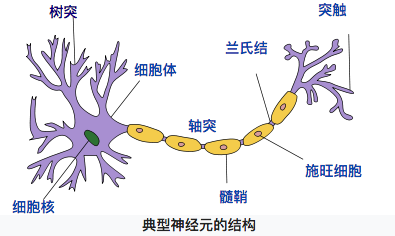【图1 典型神经元结构 （图片来自维基百科）】
神经元大致可以分为树突、突触、细胞体和轴突。树突为神经元的输入通道，其功能是将其它神经元的动作电位传递至细胞体。其它神经元的动作电位借由位于树突分支上的多个突触传递至树突上。神经细胞可以视为有两种状态的机器，激活时为“是”，不激活时为“否”。神经细胞的状态取决于从其他神经细胞接收到的信号量，以及突触的性质（抑制或加强）。当信号量超过某个阈值时，细胞体就会被激活，产生电脉冲。电脉冲沿着轴突并通过突触传递到其它神经元。（内容来自维基百科“感知机”）
同理，我们的神经元模型就是为了模拟上述过程，典型的神经元模型如下：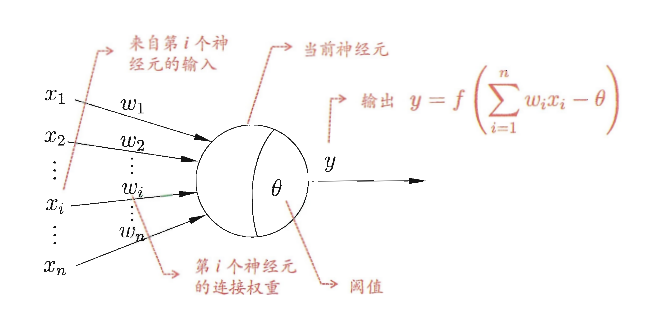【图2 典型神经元模型结构 （摘自周志华老师《机器学习》第97页）】
这个模型中，每个神经元都接受来自其它神经元的输入信号，每个信号都通过一个带有权重的连接传递，神经元把这些信号加起来得到一个总输入值，然后将总输入值与神经元的阈值进行对比（模拟阈值电位），然后通过一个“激活函数”处理得到最终的输出（模拟细胞的激活），这个输出又会作为之后神经元的输入一层一层传递下去。
1.2 神经元激活函数
本文主要介绍2种激活函数，分别是$sigmoid$和$relu$函数，函数公式如下：
$sigmoid(z)=\frac{1}{1+e^{-z}}$
$relu(z)= \left\{ \begin{array}{rcl} z & z>0\\ 0&z\leq0\end{array} \right.$
做函数图如下：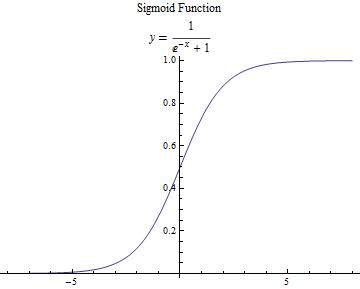$sigmoid(z)$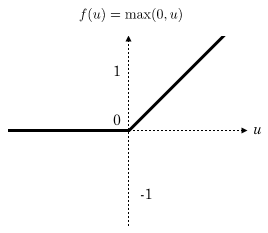$relu(z)$
【图3 激活函数】

补充说明
【补充说明的内容建议在看完后文的反向传播部分之后再回来阅读，我只是为了文章结构的统一把这部分内容添加在了这里】
引入激活函数的目的是在模型中引入非线性。如果没有激活函数，那么无论你的神经网络有多少层，最终都是一个线性映射，单纯的线性映射无法解决线性不可分问题。引入非线性可以让模型解决线性不可分问题。
一般来说，在神经网络的中间层更加建议使用$relu$函数，两个原因：

$relu$函数计算简单，可以加快模型速度；
由于反向传播过程中需要计算偏导数，通过求导可以得到$sigmoid$函数导数的最大值为0.25，如果使用$sigmoid$函数的话，每一层的反向传播都会使梯度最少变为原来的四分之一，当层数比较多的时候可能会造成梯度消失，从而模型无法收敛。

1.3 神经网络结构
我们使用如下神经网络结构来进行介绍，第0层是输入层（3个神经元）， 第1层是隐含层（2个神经元），第2层是输出层：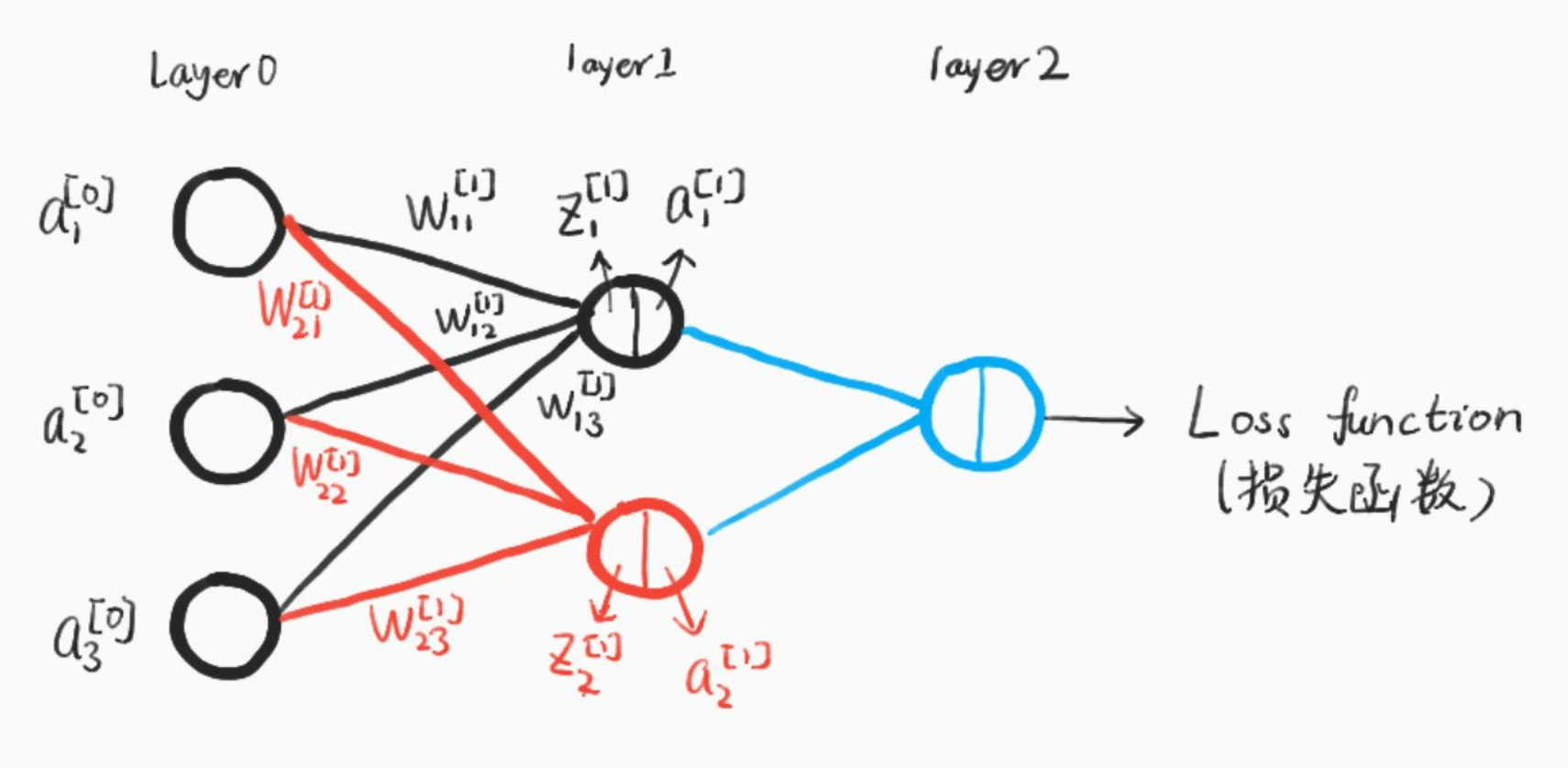【图4 神经网络结构（手绘）】
我们使用以下符号约定，$w_{jk}^{[l]}$表示从网络第$(l-1)^{th}$中$k^{th}$个神经元指向第$l^{th}$中第$j^{th}$个神经元的连接权重，比如上图中$w^{}_{21}$即从第0层第1个神经元指向第1层第2个神经元的权重。同理，我们使用$b^{[l]}_j$来表示第$l^{th}$层中第$j^{th}$神经元的偏差，用$z^{[l]}_j$来表示第$l^{th}$层中第$j^{th}$神经元的线性结果,用$a^{[l]}_j$来表示第$l^{th}$层中第$j^{th}$神经元的激活函数输出。
激活函数使用符号$\sigma$表示，因此，第$l^{th}$层中第$j^{th}$神经元的激活为：
$a^{[l]}_j=\sigma(\sum_kw^{[l]}_{jk}a^{[l-1]}_k+b^{[l]}_j)$
现在，我们使用矩阵形式重写这个公式：
定义$w^{[l]}$表示权重矩阵，它的每一个元素表示一个权重，即每一行都是连接第$l$层的权重，用上图举个例子就是：
$w^{}=\left[ \begin{array}{cc} w_{11}^{} & w_{12}^{} & w_{13}^{} \\ w_{21}^{}& w_{22}^{} & w_{23}^{}\end{array}\right]$
同理，
$b^{}=\left[ \begin{array}{cc}b^{}_1 \\ b^{}_2 \end{array}\right]$
$z^{}=\left[ \begin{array}{cc} w_{11}^{} & w_{12}^{} & w_{13}^{} \\ w_{21}^{}& w_{22}^{} & w_{23}^{}\end{array}\right]\cdot \left[ \begin{array}{cc} a^{}_1 \\ a^{}_2 \\ a^{}_3 \end{array}\right] +\left[ \begin{array}{cc}b^{}_1 \\ b^{}_2 \end{array}\right]=\left[ \begin{array}{cc} w_{11}^{}a^{}_1+w_{12}^{}a^{}_2+w_{13}^{}a^{}_3+b^{}_1 \\ w^{}_{21}a^{}_1+w_{22}^{}a^{}_2+w_{23}^{}a^{}_3+b^{}_2\end{array}\right]$
更一般地，我们可以把前向传播过程表示：
$a^{[l]}=\sigma(w^{[l]}a^{[l-1]}+b^{[l]})$
到这里，我们已经讲完了前向传播的过程，值得注意的是，这里我们只有一个输入样本，对于多个样本同时输入的情况是一样的，只不过我们的输入向量不再是一列，而是m列，每一个都表示一个输入样本。
多样本输入情况下的表示为：
$Z^{[l]}=w^{[l]}\cdot A^{[l-1]}+b^{[l]}$
$A^{[l]}=\sigma(Z^{[l]})$
其中，此时$A^{[l-1]}=\left[ \begin{array}{cc} |&|&\ldots&| \\a^{[l-1](1)}&a^{[l-1](2)}&\ldots&a^{[l-1](m)} \\ |&|&\ldots&|\end{array}\right]$
每一列都表示一个样本，从样本1到m
$w^{[l]}$的含义和原来完全一样，$Z^{[l]}$也会变成m列，每一列表示一个样本的计算结果。

之后我们的叙述都是先讨论单个样本的情况，再扩展到多个样本同时计算。

2. 损失函数和代价函数
说实话，**损失函数（Loss Function）和代价函数（Cost Function）**并没有一个公认的区分标准，很多论文和教材似乎把二者当成了差不多的东西。
为了后面描述的方便，我们把二者稍微做一下区分（这里的区分仅仅对本文适用，对于其它的文章或教程需要根据上下文自行判断含义）：

损失函数主要指的是对于单个样本的损失或误差；代价函数表示多样本同时输入模型的时候总体的误差——每个样本误差的和然后取平均值。

举个例子，如果我们把单个样本的损失函数定义为：
$L(a,y)=-[y \cdot log(a)+(1-y)\cdot log(1-a)]$
那么对于m个样本，代价函数则是：
$C=-\frac{1}{m}\sum_{i=0}^m(y^{(i)}\cdot log(a^{(i)})+(1-y^{(i)})\cdot log(1-a^{(i)}))$
3. 反向传播
反向传播的基本思想就是通过计算输出层与期望值之间的误差来调整网络参数，从而使得误差变小。
反向传播的思想很简单，然而人们认识到它的重要作用却经过了很长的时间。后向传播算法产生于1970年，但它的重要性一直到David Rumelhart，Geoffrey Hinton和Ronald Williams于1986年合著的论文发表才被重视。
事实上，人工神经网络的强大力量几乎就是建立在反向传播算法基础之上的。反向传播基于四个基础等式，数学是优美的，仅仅四个等式就可以概括神经网络的反向传播过程，然而理解这种优美可能需要付出一些脑力。事实上，反向传播如此之难，以至于相当一部分初学者很难进行独立推导。所以如果读者是初学者，希望读者可以耐心地研读本节。对于初学者，我觉得拿出1-3个小时来学习本小节是比较合适的，当然，对于熟练掌握反向传播原理的读者，你可以在十几分钟甚至几分钟之内快速浏览本节的内容。
3.1 矩阵补充知识
对于大部分理工科的研究生，以及学习过矩阵论或者工程矩阵理论相关课程的读者来说，可以跳过本节。
本节主要面向只学习过本科线性代数课程或者已经忘记矩阵论有关知识的读者。
总之，具备了本科线性代数知识的读者阅读这一小节应该不会有太大问题。本节主要在线性代数的基础上做一些扩展。（不排除少数本科线性代数课程也涉及到这些内容，如果感觉讲的简单的话，勿喷）
3.1.1 求梯度矩阵
假设函数  $f:R^{m\times n}\rightarrow R$可以把输入矩阵（shape: $m\times n$）映射为一个实数。那么，函数$f$的梯度定义为：
$\nabla_Af(A)=\left[ \begin{array}{cc} \frac{\partial f(A)}{\partial A_{11}}&\frac{\partial f(A)}{\partial A_{12}}&\ldots&\frac{\partial f(A)}{\partial A_{1n}} \\ \frac{\partial f(A)}{\partial A_{21}}&\frac{\partial f(A)}{\partial A_{22}}&\ldots&\frac{\partial f(A)}{\partial A_{2n}} \\\vdots &\vdots &\ddots&\vdots\\ \frac{\partial f(A)}{\partial A_{m1}}&\frac{\partial f(A)}{\partial A_{m2}}&\ldots&\frac{\partial f(A)}{\partial A_{mn}}\end{array}\right]$
即$(\nabla_Af(A))_{ij}=\frac{\partial f(A)}{\partial A_{ij}}$
同理，一个输入是向量（向量一般指列向量，本文在没有特殊声明的情况下默认指的是列向量）的函数$f:R^{n\times 1}\rightarrow R$，则有：
$\nabla_xf(x)=\left[ \begin{array}{cc}\frac{\partial f(x)}{\partial x_1}\\ \frac{\partial f(x)}{\partial x_2}\\ \vdots \\ \frac{\partial f(x)}{\partial x_n} \end{array}\right]$

注意：这里涉及到的梯度求解的前提是函数$f$ 返回的是一个实数，如果函数返回的是一个矩阵或者向量，那么我们是没有办法求梯度的。比如，对函数$f(A)=\sum_{i=0}^m\sum_{j=0}^nA_{ij}^2$，由于返回一个实数,我们可以求解梯度矩阵。如果$f(x)=Ax (A\in R^{m\times n}, x\in R^{n\times 1})$，由于函数返回一个$m$行1列的向量，因此不能对$f$求梯度矩阵。

根据定义，很容易得到以下性质：

$\nabla_x(f(x)+g(x))=\nabla_xf(x)+\nabla_xg(x)$
$\nabla(tf(x))=t\nabla f(x), t\in R$

有了上述知识，我们来举个例子：

定义函数$f:R^m\rightarrow R, f(z)=z^Tz$,那么很容易得到$\nabla_zf(z)=2z$，具体请读者自己证明。

3.1.2 海塞矩阵
定义一个输入为$n$维向量，输出为实数的函数$f:R^n\rightarrow R$，那么海塞矩阵（Hessian Matrix）定义为多元函数$f$的二阶偏导数构成的方阵：
$\nabla^2_xf(x)=\left[ \begin{array}{cc} \frac{\partial^2f(x)}{\partial x_1^2}&\frac{\partial^2f(x)}{\partial x_1\partial x_2}&\ldots &\frac{\partial^2f(x)}{\partial x_1\partial x_n}\\ \frac{\partial^2f(x)}{\partial x_2\partial x_1}&\frac{\partial^2f(x)}{\partial x_2^2}&\ldots&\frac{\partial^2f(x)}{\partial x_2\partial x_n}\\ \vdots&\vdots&\ddots&\vdots\\\frac{\partial^2f(x)}{\partial x_n\partial x_1}&\frac{\partial^2f(x)}{\partial x_n\partial x_2}&\ldots&\frac{\partial^2f(x)}{\partial x_n^2}\end{array}\right]$
由上式可以看出，海塞矩阵总是对称阵。

注意：很多人把海塞矩阵看成$\nabla _xf(x)$的导数，这是不对的。只能说，海塞矩阵的每个元素都是函数$f$二阶偏导数。那么，有什么区别呢？
首先，来看正确的解释。**海塞矩阵的每个元素是函数$f$的二阶偏导数。**拿$\frac{\partial^2f(x)}{\partial x_1\partial x_2}$举个例子，函数$f$对$x_1$求偏导得到的是一个实数，比如$\frac{\partial^2f(x)}{\partial x_1}=x_2^3x_1$，因此继续求偏导是有意义的,继续对$x_2$求偏导可以得到$3x_1x_2^2$。
然后,来看一下错误的理解。把海塞矩阵看成$\nabla _xf(x)$的导数，也就是说错误地以为$\nabla^2_xf(x)=\nabla_x(\nabla_xf(x))$，要知道，$\nabla_xf(x)$是一个向量，而在上一小节我们已经重点强调过，在我们的定义里对向量求偏导是没有定义的。
但是$\nabla_x\frac{\partial f(x)}{\partial x_i}$是有意义的，因为$\frac{\partial f(x)}{\partial x_i}$是一个实数，具体地：
$\nabla_x\frac{\partial f(x)}{\partial x_i}=\left[ \begin{array}{cc} \frac{\partial^2f(x)}{\partial x_i\partial x_1}\\\frac{\partial^2f(x)}{\partial x_i\partial x_2}\\\vdots\\\frac{\partial^2f(x)}{\partial x_i\partial x_n}\end{array}\right]$
即海塞矩阵的第i行（或列）。
希望读者可以好好区分。

3.1.3 总结
根据3.1.1和3.1.2小节的内容很容易得到以下等式：

$b\in R^{n}, x\in R^n, A\in R^{n\times n}并且A 是对称矩阵$
$b,x$均为列向量
那么，
$\nabla_xb^Tx=b$
$\nabla_xx^TAx=2Ax(A是对称阵)$
$\nabla^2_xx^TAx=2A(A是对称阵)$
这些公式可以根据前述定义自行推导，有兴趣的读者可以自己推导一下。
####3.2 矩阵乘积和对应元素相乘
在下一节讲解反向传播原理的时候，尤其是把公式以矩阵形式表示的时候，需要大家时刻区分什么时候需要矩阵相乘，什么时候需要对应元素相乘。

比如对于矩阵$A=\left[ \begin{array}{cc} 1&2\\3&4\end{array}\right]，矩阵B=\left[ \begin{array}{cc} -1&-2\\-3&-4\end{array}\right]$
矩阵相乘
$AB=\left[\begin{array}{cc}1\times -1+2\times -3&1\times -2+2\times -4\\3\times -1+4\times -3&3\times -2+4\times -4\end{array}\right]=\left[\begin{array}{cc}-7&-10\\-15&-22\end{array}\right]$
对应元素相乘使用符号$\odot$表示：
$A\odot B=\left[\begin{array}{cc}1\times -1&2\times -2 \\ 3\times -3&4\times -4\end{array}\right]=\left[\begin{array}{cc}-1&-4 \\ -9&-16\end{array}\right]$
3.3 梯度下降法原理
通过之前的介绍，相信大家都可以自己求解梯度矩阵（向量）了。
那么梯度矩阵（向量）求出来的意义是什么？从几何意义讲，梯度矩阵代表了函数增加最快的方向，因此，沿着与之相反的方向就可以更快找到最小值。如图5所示：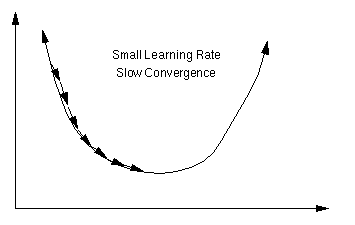【图5 梯度下降法 图片来自百度】

反向传播的过程就是利用梯度下降法原理，慢慢的找到代价函数的最小值，从而得到最终的模型参数。梯度下降法在反向传播中的具体应用见下一小节。
3.4 反向传播原理（四个基础等式）
反向传播能够知道如何更改网络中的权重$w$ 和偏差$b$ 来改变代价函数值。最终这意味着它能够计算偏导数$\frac{\partial L(a^{[l]},y)} {\partial w^{[l]}_{jk}}$ 和$\frac{\partial L(a^{[l]},y)}{\partial b^{[l]}_j}$
为了计算这些偏导数，我们首先引入一个中间变量$\delta^{[l]}_j$，我们把它叫做网络中第$l^{th}$层第$j^{th}$个神经元的误差。后向传播能够计算出误差$\delta^{[l]}_j$，然后再将其对应回$\frac{\partial L(a^{[l]},y)}{\partial w^{[l]}_{jk}}$和$\frac{\partial L(a^{[l]},y)}{\partial b^{[l]}_j}$ 。
那么，如何定义每一层的误差呢？如果为第$l$ 层第$j$ 个神经元添加一个扰动$\Delta z^{[l]}_j$，使得损失函数或者代价函数变小，那么这就是一个好的扰动。通过选择 $\Delta z^{[l]}_j$与$\frac{\partial L(a^{[l]}, y)}{\partial z^{[l]}_j}$符号相反（梯度下降法原理），就可以每次都添加一个好的扰动最终达到最优。
受此启发，我们定义网络层第$l$ 层中第$j$ 个神经元的误差为$\delta^{[l]}_j$:
$\delta^{[l]}_j=\frac{\partial L(a^{[L], y})}{\partial z^{[l]}_j}$
于是，每一层的误差向量可以表示为：
$\delta ^{[l]}=\left[\begin{array}{cc}\delta ^{[l]}_1\\\delta ^{[l]}_2\\ \vdots \\ \delta ^{[l]}_n\end{array} \right]$
下面开始正式介绍四个基础等式【确切的说是四组等式】

**注意：**这里我们的输入为单个样本(所以我们在下面的公式中使用的是损失函数而不是代价函数)。多个样本输入的公式会在介绍完单个样本后再介绍。

等式1 ：输出层误差

$\delta^{[L]}_j=\frac{\partial L}{\partial a^{[L]}_j}\sigma^{'}(z^{[L]}_j)$
其中，$L$表示输出层层数。以下用$\partial L$ 表示 $\partial L(a^{[L]}, y)$
写成矩阵形式是：
$\delta^{[L]}=\nabla _aL\odot \sigma^{'}(z^{[L]})$
【注意是对应元素相乘，想想为什么？】

说明
根据本小节开始时的叙述，我们期望找到$\partial L \ /\partial z^{[l]}_j$，然后朝着方向相反的方向更新网络参数，并定义误差为：
$\delta^{[L]}_j=\frac{\partial L}{\partial z^{[L]}_j}$
根据链式法则，
$\delta^{[L]}_j = \sum_k \frac{\partial L}{\partial a^{[L]}_k} \frac{\partial a^{[L]}_k}{\partial z^{[L]}_j}$
当$k\neq j$时，$\partial a^{[L]}_k / \partial z^{[L]}_j$就为零。结果我们可以简化之前的等式为
$\delta^{[L]}_j = \frac{\partial L}{\partial a^{[L]}_j} \frac{\partial a^{[L]}_j}{\partial z^{[L]}_j}$
重新拿出定义：$a^{[L]}_j = \sigma(z^{[L]}_j)$，就可以得到：
$\delta^{[L]}_j = \frac{\partial L}{\partial a^{[L]}_j} \sigma'(z^{[L]}_j)$
再"堆砌"成向量形式就得到了我们的矩阵表示式（这也是为什么使用矩阵形式表示需要 对应元素相乘 的原因）。

等式2： 隐含层误差
$\delta^{[l]}_j = \sum_k w^{[l+1]}_{kj} \delta^{[l+1]}_k \sigma'(z^{[l]}_j)$

写成矩阵形式：
$\delta^{[l]}=[w^{[l+1]T}\delta^{[l+1]}]\odot \sigma ^{'}(z^{[l]})$

说明：
$z^{[l+1]}_k=\sum_jw^{[l+1]}_{kj}a^{[l]}_j+b^{[l+1]}_k=\sum_jw^{[l+1]}_{kj}\sigma(z^{[l]}_j)+b^{[l+1]}_k$
进行偏导可以获得：
$\frac{\partial z^{[l+1]}_k}{\partial z^{[l]}_j} = w^{[l+1]}_{kj} \sigma'(z^{[l]}_j)$
代入得到：
$\delta^{[l]}_j = \sum_k w^{[l+1]}_{kj} \delta^{[l+1]}_k \sigma'(z^{[l]}_j)$

等式3：参数变化率

$\frac{\partial L}{\partial b^{[l]}_j}=\delta^{[l]}_j$
$\frac{\partial L}{\partial w^{[l]}_{jk}}=a^{[l-1]}_k\delta^{[l]}_j$
写成矩阵形式：
$\frac{\partial L}{\partial b^{[l]}}=\delta^{[l]}$$\frac{\partial L}{\partial w^{[l]}}=\delta^{[l]}a^{[l-1]T}$

说明：
根据链式法则推导。
由于
$z^{[l]}_j=\sum_kw^{[l]}_{jk}a^{[l]}_k+b^{[l]}_k$
对$b^{[l]}_j$求偏导得到：
$\frac{\partial L}{\partial b^{[l]}_j}=\frac{\partial L}{\partial z^{[l]}_j}\frac{\partial z^{[l]}_j}{b^{[l]}_j}=\delta^{[l]}_j$
对$w^{[l]}_{jk}$求偏导得到：
$\frac{\partial L}{\partial w^{[l]}_{jk}}=\frac{\partial L}{\partial z^{[l]}_j}\frac{\partial z^{[l]}_j}{w^{[l]}_{jk}}=a^{[l-1]}_k\delta^{[l]}_j$
最后再变成矩阵形式就好了。

对矩阵形式来说，需要特别注意维度的匹配。强烈建议读者在自己编写程序之前，先列出这些等式，然后仔细检查维度是否匹配。

很容易看出$\frac{\partial L}{\partial w^{[l]}}$是一个$dim(\delta^{[l]})$行$dim(a^{[l-1]})$列的矩阵，和$w^{[l]}$的维度一致；$\frac{\partial L}{\partial b^{[l]}}$是一个维度为$dim(\delta^{[l]})$的列向量

等式4：参数更新规则

这应该是这四组公式里最简单的一组了，根据梯度下降法原理，朝着梯度的反方向更新参数：
$b^{[l]}_j\leftarrow b^{[l]}_j-\alpha \frac{\partial L}{\partial b^{[l]}_j}$
$w^{[l]}_{jk}\leftarrow w^{[l]}_{jk}-\alpha\frac{\partial L}{\partial w^{[l]}_{jk}}$
写成矩阵形式：
$b^{[l]}\leftarrow b^{[l]}-\alpha\frac{\partial L}{\partial b^{[l]}}$
$w^{[l]}\leftarrow w^{[l]}-\alpha\frac{\partial L}{\partial w^{[l]}}$

这里的$\alpha$指的是学习率。学习率指定了反向传播过程中梯度下降的步长。

3.5 反向传播总结
我们可以得到如下最终公式：
3.5.1 单样本输入公式表

说明
公式
备注

输出层误差
$\delta^{[L]}=\nabla _aL\odot \sigma^{'}(z^{[L]})$

隐含层误差
$\delta^{[l]}=[w^{[l+1]T}\delta^{[l+1]}]\odot \sigma ^{'}(z^{[l]})$

参数变化率
$\frac{\partial L}{\partial b^{[l]}}=\delta^{[l]}$$\frac{\partial L}{\partial w^{[l]}}=\delta^{[l]}a^{[l-1]T}$
注意维度匹配

参数更新
$b^{[l]}\leftarrow b^{[l]}-\alpha\frac{\partial L}{\partial b^{[l]}}$$w^{[l]}\leftarrow w^{[l]}-\alpha\frac{\partial L}{\partial w^{[l]}}$
$\alpha$是学习率

3.5.2 多样本输入公式表
多样本：需要使用代价函数，如果有m个样本，那么由于代价函数有一个$\frac{1}{m}$的常数项，因此所有的参数更新规则都需要有一个$\frac{1}{m}$的前缀。
多样本同时输入的时候需要格外注意维度匹配，一开始可能觉得有点混乱，但是不断加深理解就会豁然开朗。

说明
公式
备注

输出层误差
$dZ^{[L]}=\nabla _AC\odot \sigma^{'}(Z^{[L]})$
此时$dZ^{[l]}$不再是一个列向量，变成了一个$m$列的矩阵，每一列都对应一个样本的向量

隐含层误差
$dZ^{[l]}=[w^{[l+1]T}dZ^{[l+1]}]\odot \sigma ^{'}(Z^{[l]})$
此时$dZ^{[l]}$的维度是$n\times m$，$n$表示第l层神经元的个数，m表示样本数

参数变化率
$db^{[l]}=\frac{\partial C}{\partial b^{[l]}}=\frac{1}{m}meanOfEachRow(dZ^{[l]})\\dw^{[l]}=\frac{\partial C}{\partial w^{[l]}}=\frac{1}{m}dZ^{[l]}A^{[l-1]T}$
更新$b^{[l]}$的时候需要对每行求均值；   注意维度匹配;   $m$是样本个数

参数更新
$b^{[l]}\leftarrow b^{[l]}-\alpha\frac{\partial C}{\partial b^{[l]}}$$w^{[l]}\leftarrow w^{[l]}-\alpha\frac{\partial C}{\partial w^{[l]}}$
$\alpha$是学习率

3.5.3 关于超参数
通过前面的介绍，相信读者可以发现BP神经网络模型有一些参数是需要设计者给出的，也有一些参数是模型自己求解的。
那么，哪些参数是需要模型设计者确定的呢？
比如，学习率$\alpha$，隐含层的层数，每个隐含层的神经元个数，激活函数的选取，损失函数（代价函数）的选取等等，这些参数被称之为超参数。
其它的参数，比如权重矩阵$w$和偏置系数$b$在确定了超参数之后是可以通过模型的计算来得到的，这些参数称之为普通参数，简称参数。
超参数的确定其实是很困难的。因为你很难知道什么样的超参数会让模型表现得更好。比如，学习率太小可能造成模型收敛速度过慢，学习率太大又可能造成模型不收敛；再比如，损失函数的设计，如果损失函数设计不好的话，可能会造成模型无法收敛；再比如，层数过多的时候，如何设计网络结构以避免梯度消失和梯度爆炸……
神经网络的程序比一般程序的调试难度大得多，因为它并不会显式报错，它只是无法得到你期望的结果，作为新手也很难确定到底哪里出了问题（对于自己设计的网络，这种现象尤甚，我目前也基本是新手，所以这些问题也在困扰着我）。当然，使用别人训练好的模型来微调看起来是一个捷径……
总之，神经网络至少在目前来看感觉还是黑箱的成分居多，希望通过大家的努力慢慢探索吧。
4. 是不是猫？
本小节主要使用上述公式来完成一个小例子，这个小小的神经网络可以告诉我们一张图片是不是猫。本例程参考了coursera的作业，有改动。
在实现代码之前，先把用到的公式列一个表格吧，这样对照着看大家更清晰一点(如果你没有2个显示器建议先把这些公式抄写到纸上，以便和代码对照)：

编号
公式
备注

1
$Z^{[l]}=w^{[l]}A^{[l-1]}+b^{[l]}$

2
$A^{[l]}=\sigma(Z^{[l]})$

3
$dZ^{[L]}=\nabla_AC\odot\sigma^{'}(Z^{[L]})$

4
$dZ^{[l]}=[w^{[l+1]T}dZ^{[l+1]}]\odot \sigma ^{'}(Z^{[l]})$

5
$db^{[l]}=\frac{\partial C}{\partial b^{[l]}}=\frac{1}{m}meanOfEachRow(dZ^{[l]})$

6
$dw^{[l]}=\frac{\partial C}{\partial w^{[l]}}=\frac{1}{m}dZ^{[l]}A^{[l-1]T}$

7
$b^{[l]}\leftarrow b^{[l]}-\alpha \cdot db^{[l]}$

8
$w^{[l]}\leftarrow w^{[l]}-\alpha\cdot dw^{[l]}$

9
$dA^{[l]}=w^{[l]T}\odot dZ^{[l]}$

准备工作做的差不多了，让我们开始吧？等等，好像我们还没有定义代价函数是什么？OMG！好吧，看来我们得先把这个做好再继续了。
那先看结果吧，我们的代价函数是：
$C =-\frac{1}{m} \sum^{m}_{i=1}(y^{(i)}log(a^{[L](i)})+(1-y^{(i)})log(1-a^{[L](i)}))$
其中，$m$是样本数量；
下面简单介绍一下这个代价函数是怎么来的（作者非数学专业，不严谨的地方望海涵）。
.
代价函数的确定用到了统计学中的**“极大似然法”**，既然这样，那就不可避免地要介绍一下“极大似然法”了。极大似然法简单来说就是“在模型已定，参数未知的情况下，根据结果估计模型中参数的一种方法"，换句话说，极大似然法提供了一种给定观察数据来评估模型参数的方法。
举个例子（本例参考了知乎相关回答），一个不透明的罐子里有黑白两种球（球仅仅颜色不同，大小重量等参数都一样）。有放回地随机拿出一个小球，记录颜色。重复10次之后发现7次是黑球，3次是白球。问你罐子里白球的比例？
相信很多人可以一口回答“30%”，那么，为什么呢？背后的原理是什么呢？
这里我们把每次取出一个球叫做一次抽样，把“抽样10次，7次黑球，3次白球”这个事件发生的概率记为$P(事件结果|Model)$，我们的Model需要一个参数$p$表示白球的比例。那么$P(事件结果|Model)=p^3(1-p)^7$。
好了，现在我们已经有事件结果的概率公式了，接下来求解模型参数$p$，根据极大似然法的思想，既然这个事件发生了，那么为什么不让这个事件（抽样10次，7次黑球，3次白球）发生的概率最大呢？因为显然概率大的事件发生才是合理的。于是就变成了求解$p^3(1-p)^7$取最大值的$p$，即导数为0，经过求导：
$d(p^3(1-p)^7)=3p^2(1-p)^7-7p^3(1-p)^6=p^2(1-p)^6(3-10p)=0$
求解可得$p=0.3$
极大似然法有一个重要的假设：

假设所有样本独立同分布！！！

好了，现在来看看我们的神经网络模型。
最后一层我们用sigmoid函数求出一个激活输出a，如果a大于0.5，就表示这个图片是猫（$y=1$），否则就不是猫（$y=0$）。因此:
$P(y=1|x;\theta)=a$
$P(y=0|x;\theta)=1-a$

公式解释：
上述第一个公式表示，给定模型参数$\theta$和输入$x$，是猫的概率是$P(y=1|x;\theta)=a$

把两个公式合并成一个公式，即
$p(y|x;\theta)=a^y(1-a)^{(1-y)}$

这里的$\theta$指的就是我们神经网络的权值参数和偏置参数。

那么似然函数
$L(\theta)=p(Y|X;\theta)=\prod^m_{i=1}p(y^{(i)}|x^{(i)};\theta)=\prod^m_{i=1}(a^{[L](i)})^{y^{(i)}}(1-a^{[L](i)})^{(1-y^{(i)})}$
变成对数形式：
$log(L(\theta))=\sum^m_{i=1}(y^{(i)}log(a^{[L](i)})+(1-y^{(i)})log(1-a^{[L](i)}))$
所以我们的目标就是最大化这个对数似然函数，也就是最小化我们的代价函数：
$C =-\frac{1}{m} \sum^{m}_{i=1}(y^{(i)}log(a^{[L](i)})+(1-y^{(i)})log(1-a^{[L](i)}))$
其中，$m$是样本数量；
好了，终于可以开始写代码了，码字手都有点酸了，不得不说公式真的好难打。

由于代码比较简单就没有上传github。本文代码和数据文件可以在这里下载:  https://pan.baidu.com/s/1q_PzaCSXOhRLOJVF5-vy2Q，密码： d7vx
其他下载源：

4.1 辅助函数
辅助函数主要包括激活函数以及激活函数的反向传播过程函数：
其中，激活函数反向传播代码对应公式4和9.
def sigmoid(z):
"""
使用numpy实现sigmoid函数

参数：
Z numpy array
输出：
A 激活值（维数和Z完全相同）
"""
return 1/(1 + np.exp(-z))

def relu(z):
"""
线性修正函数relu

参数：
z numpy array
输出：
A 激活值（维数和Z完全相同）

"""
return np.array(z>0)*z

def sigmoidBackward(dA, cacheA):
"""
sigmoid的反向传播

参数：
dA 同层激活值
cacheA 同层线性输出
输出：
dZ 梯度

"""
s = sigmoid(cacheA)
diff = s*(1 - s)
dZ = dA * diff
return dZ

def reluBackward(dA, cacheA):
"""
relu的反向传播

参数：
dA 同层激活值
cacheA 同层线性输出
输出：
dZ 梯度

"""
Z = cacheA
dZ = np.array(dA, copy=True)
dZ[Z <= 0] = 0
return dZ

另外一个重要的辅助函数是数据读取函数和参数初始化函数：
def loadData(dataDir):
"""
导入数据

参数：
输出：
训练集，测试集以及标签
"""
train_set_y_orig = train_set_y_orig.reshape((1, train_set_y_orig.shape